# Concise Selina Solutions for Class 9 Maths Chapter 3-Compound Interest [Using Formula]

The Selina solutions for the questions given in Chapter 3, Compound Interest [Using Formula], of the Class 9 Selina textbooks are available here. In this chapter students learn about the topic of Compound Interest in detail. Students can easily score full marks in the exams by solving all the questions present in the Selina textbook.

The Class 9 Selina solutions maths are very easy to understand. These solutions cover all the exercise questions included in the book and are according to the syllabus prescribed by the ICSE or CISCE. Here, the PDF of the Class 9 maths Chapter 3 Selina solutions is available which can be downloaded as well as viewed online. Students can also avail these Selina solutions and download it for free to practice them offline as well.

### Access Answers of Maths Selina Class 9 Chapter 3- Compound Interest [Using Formula]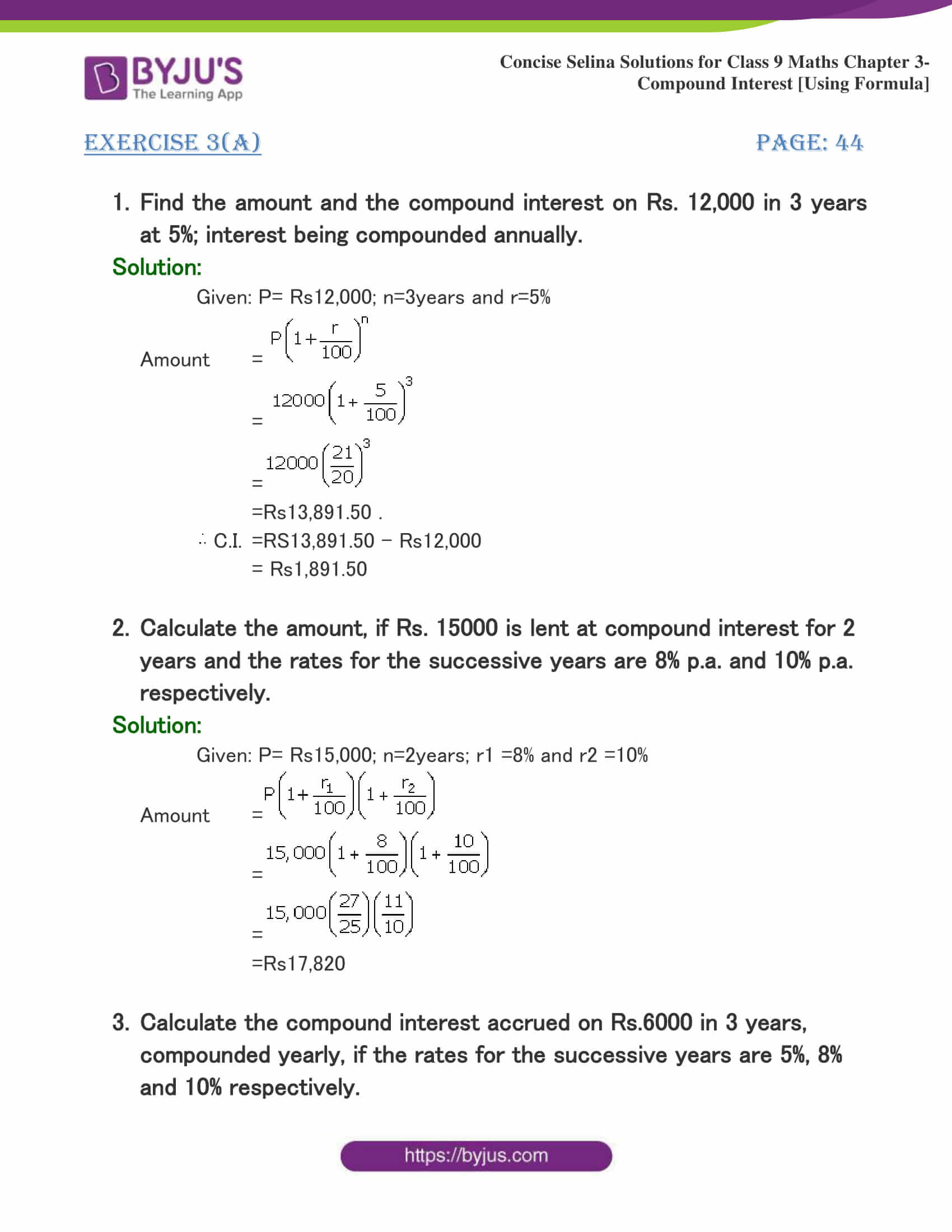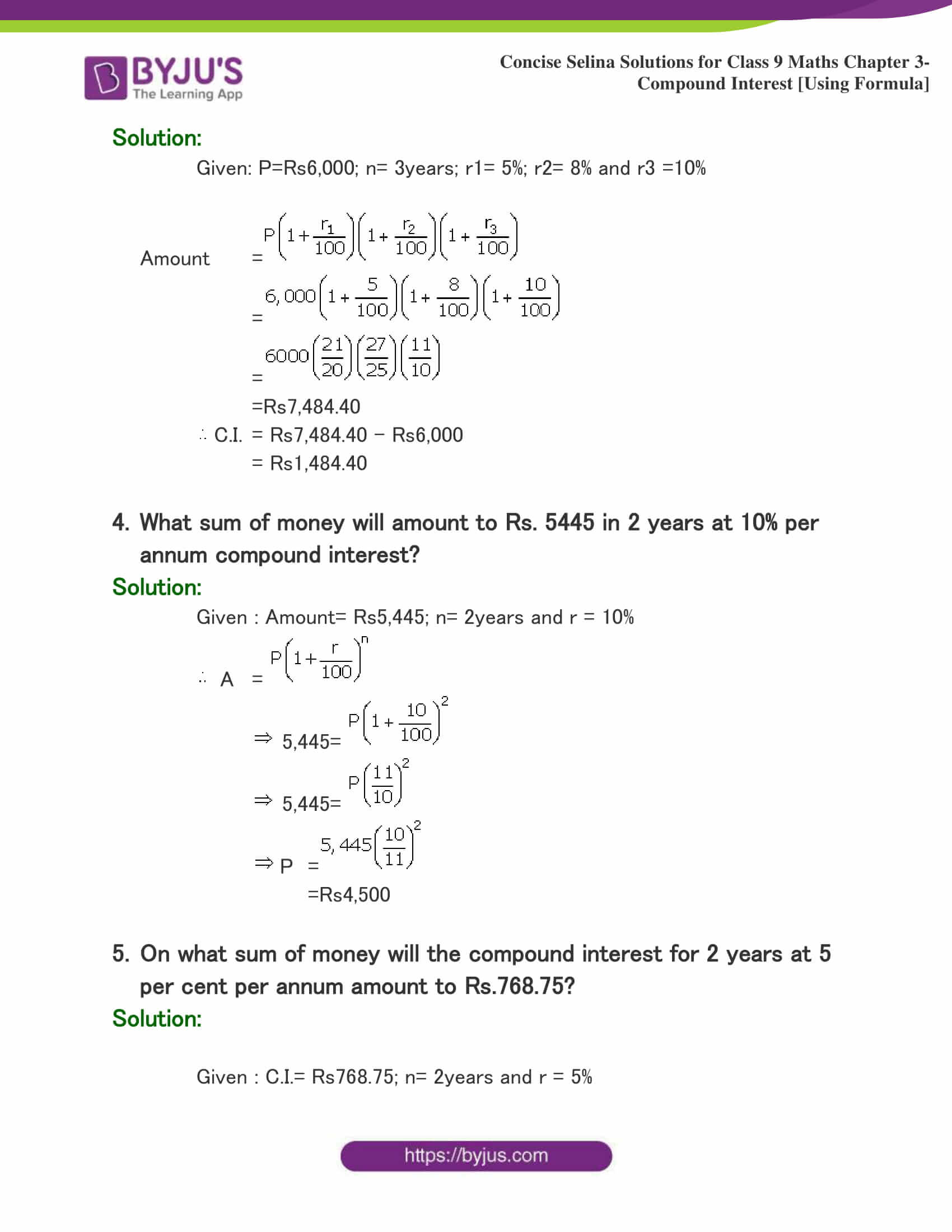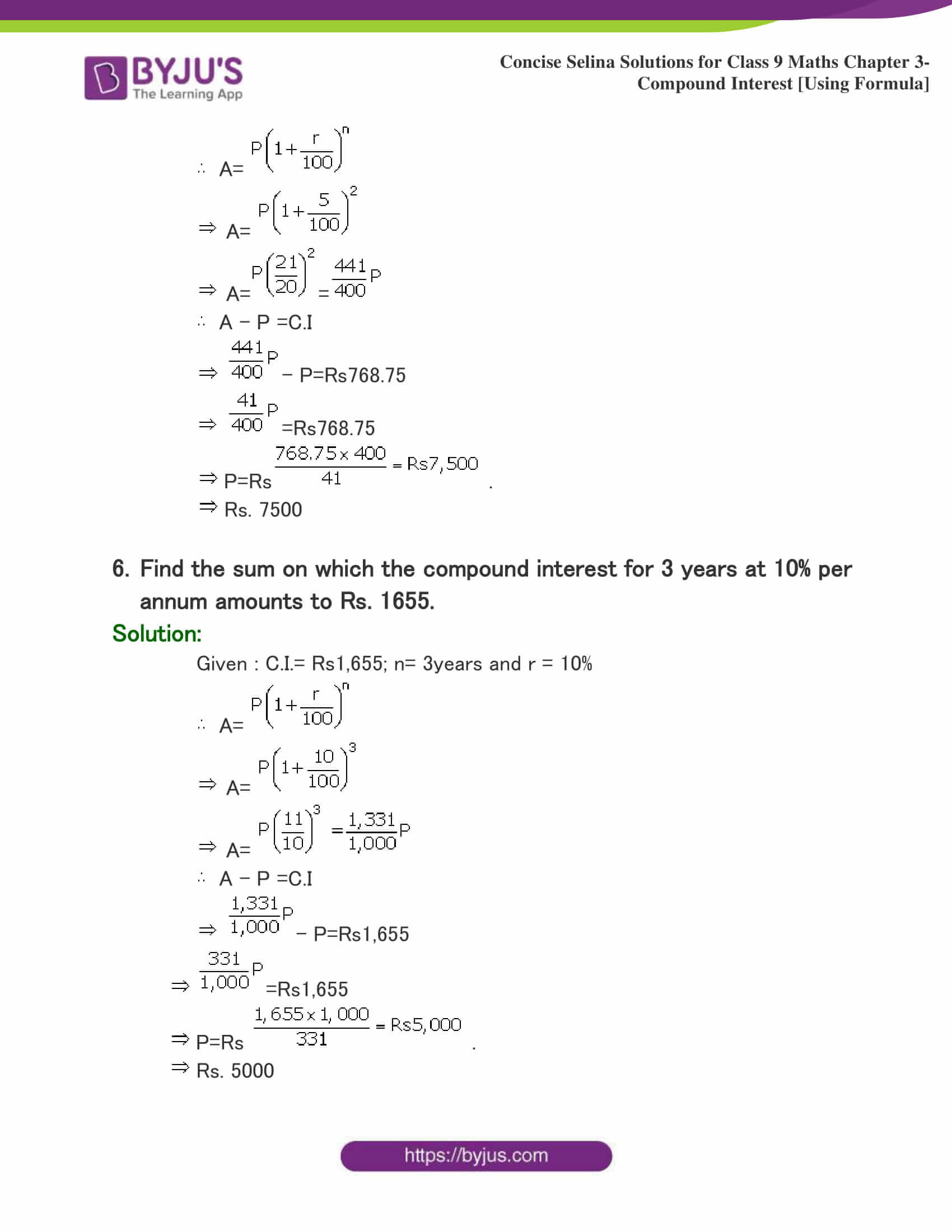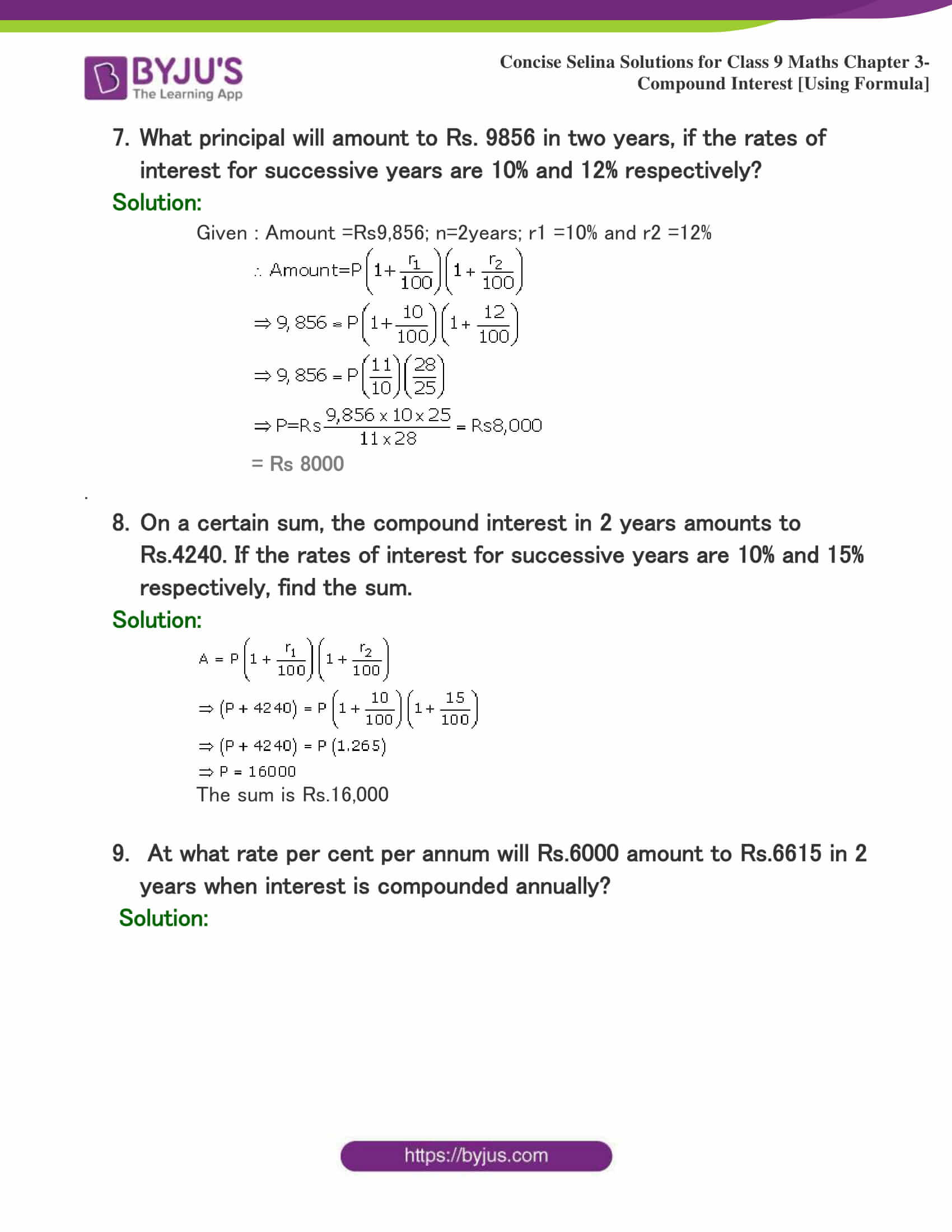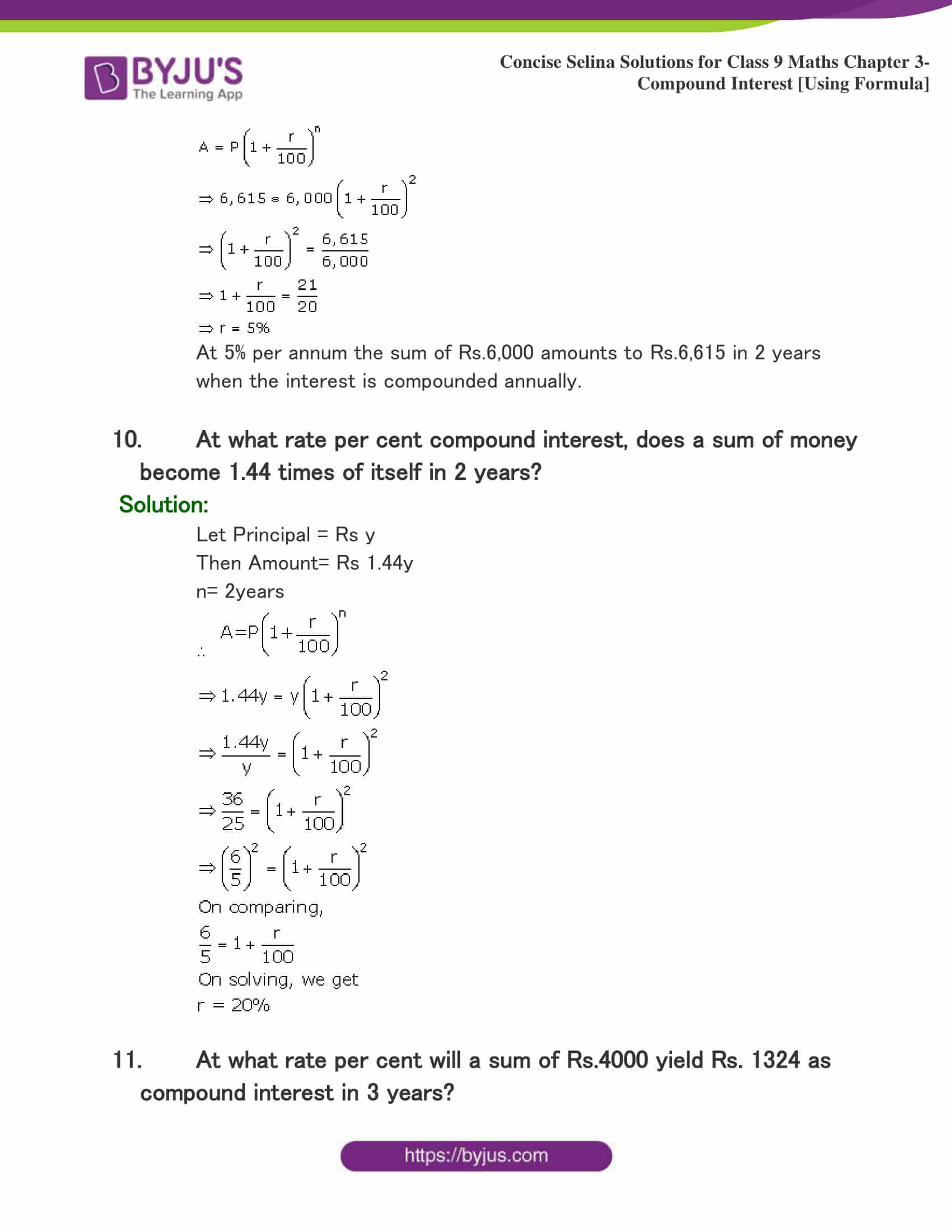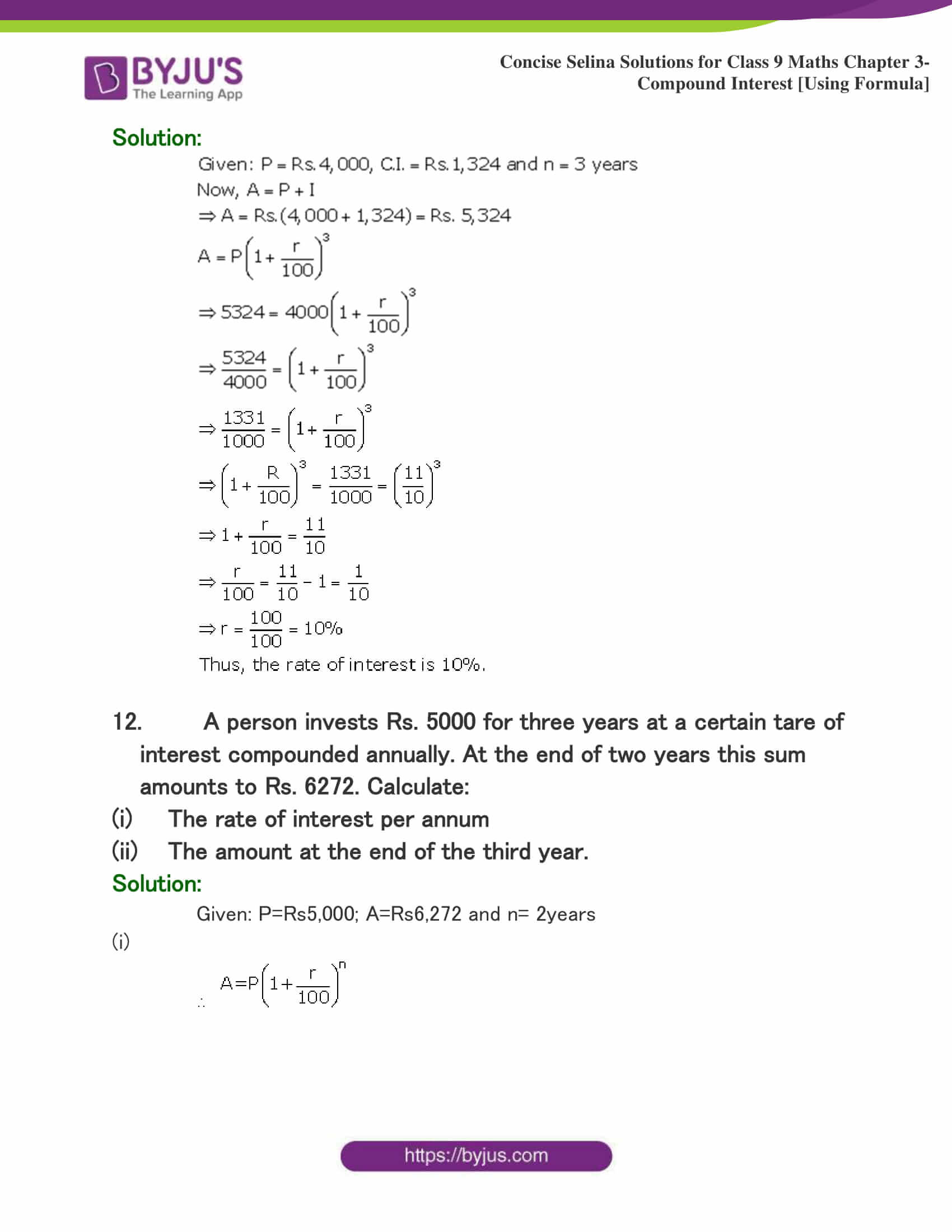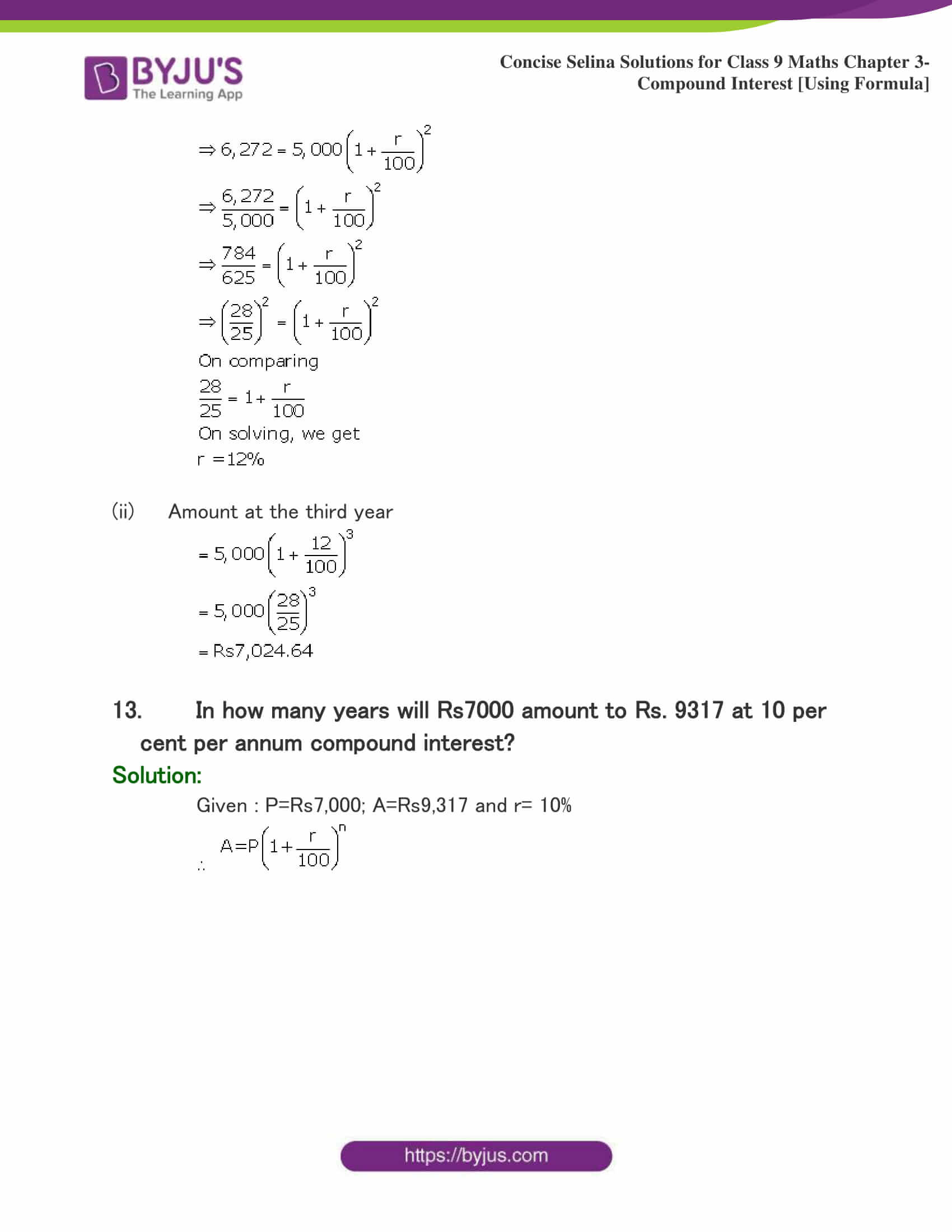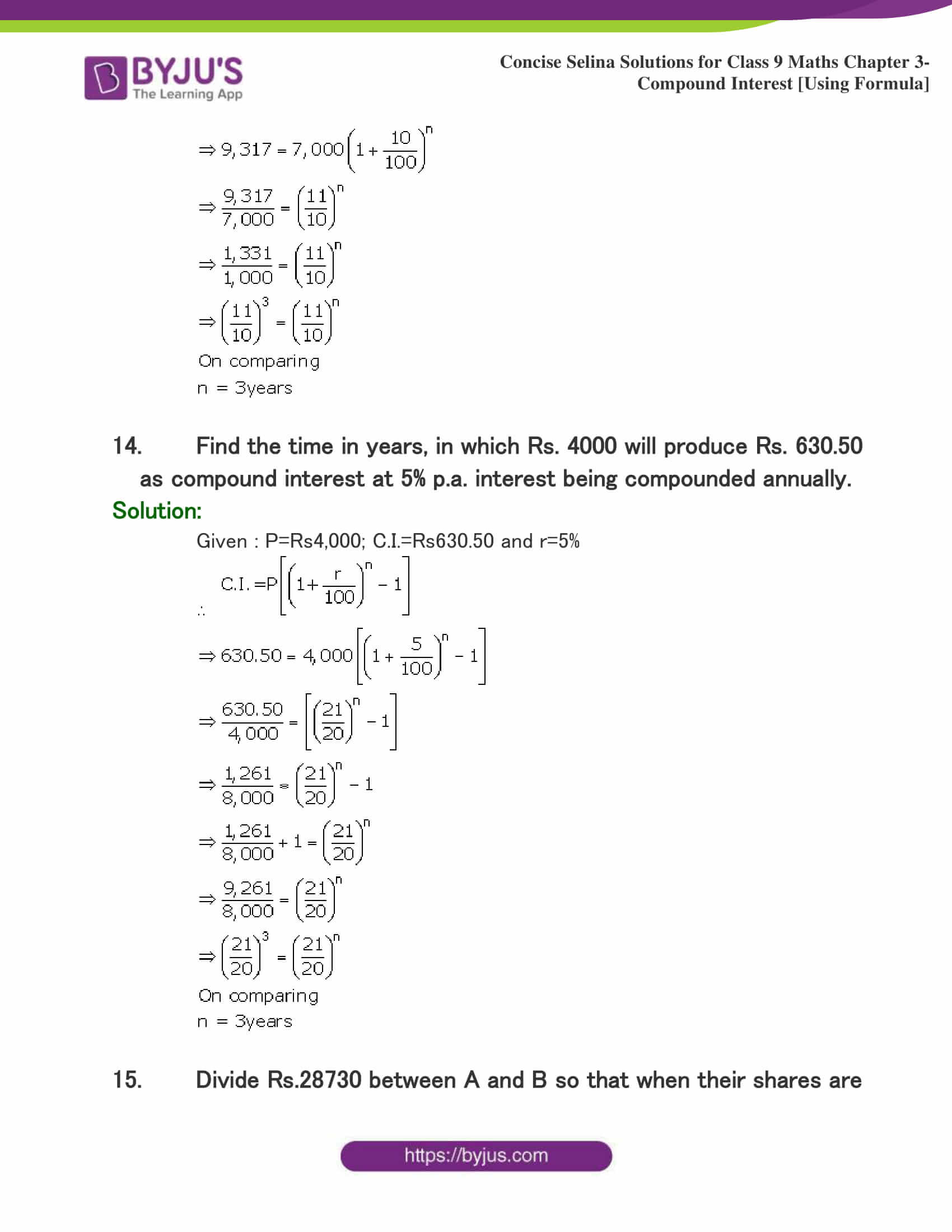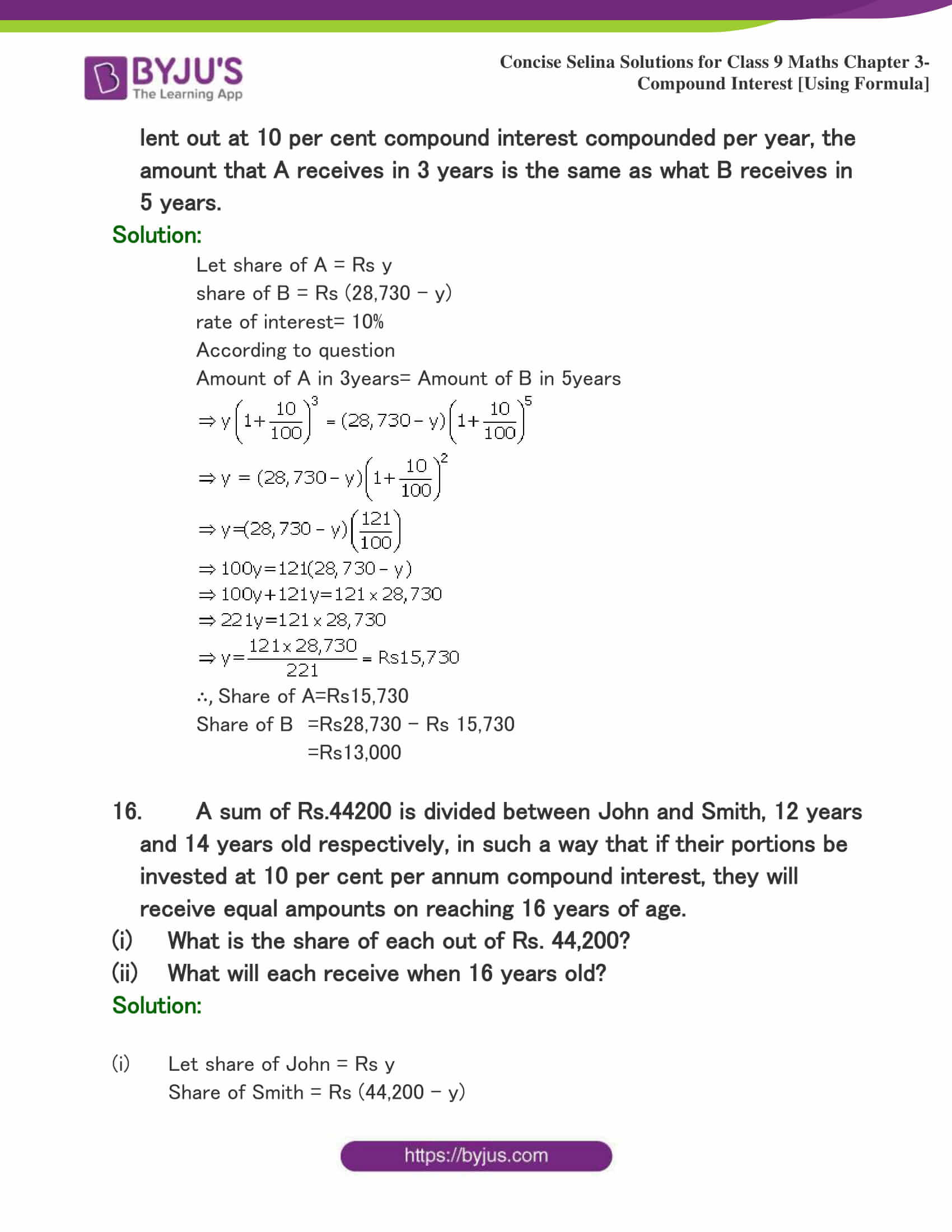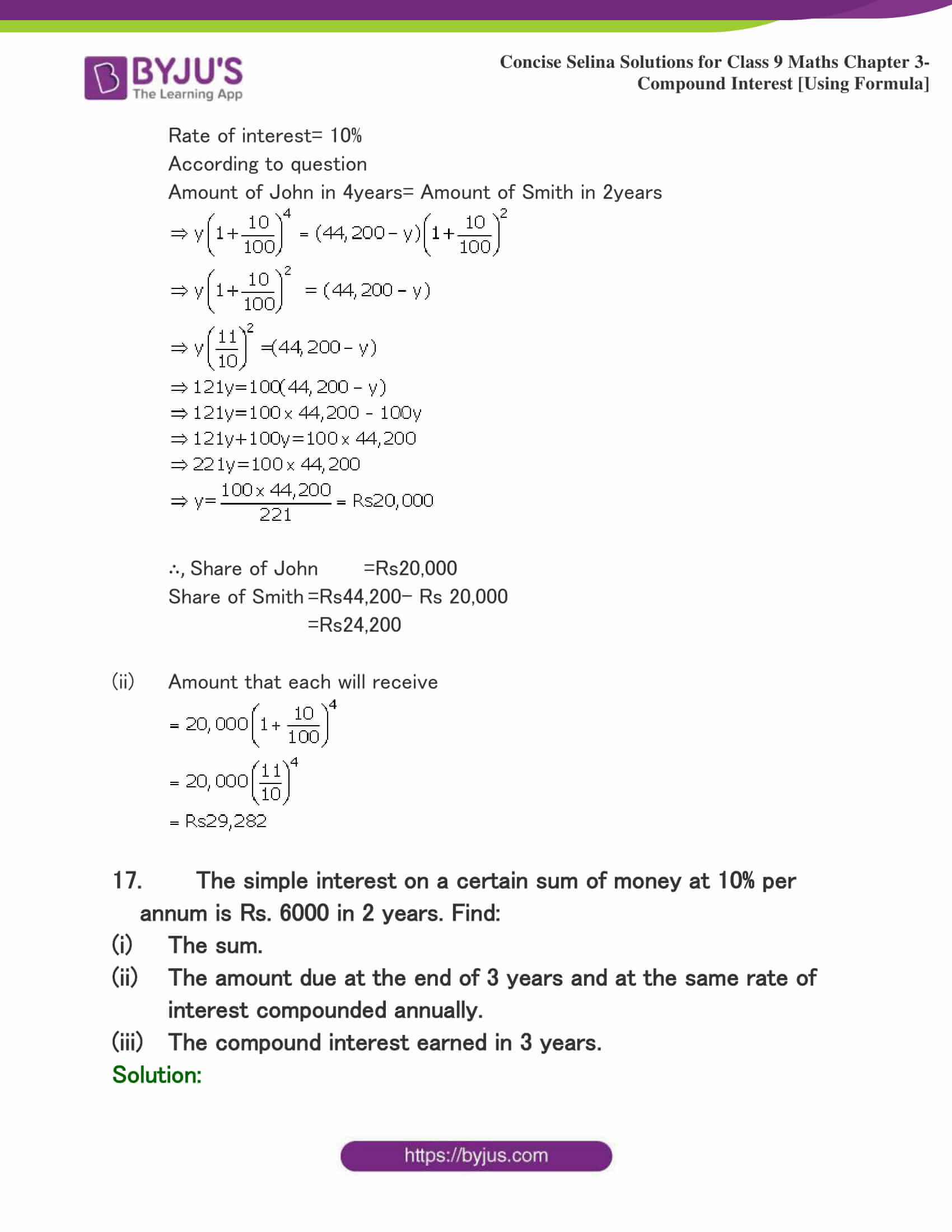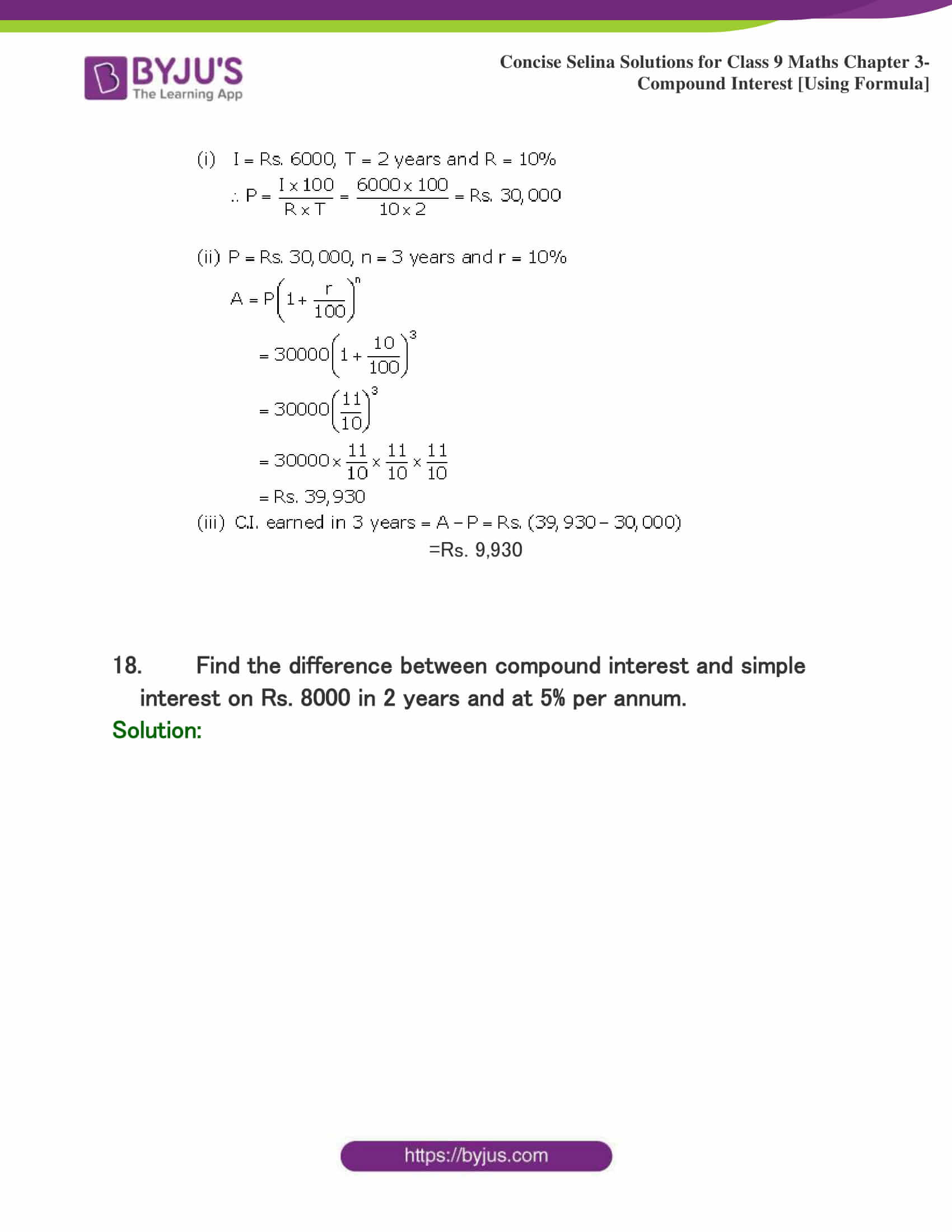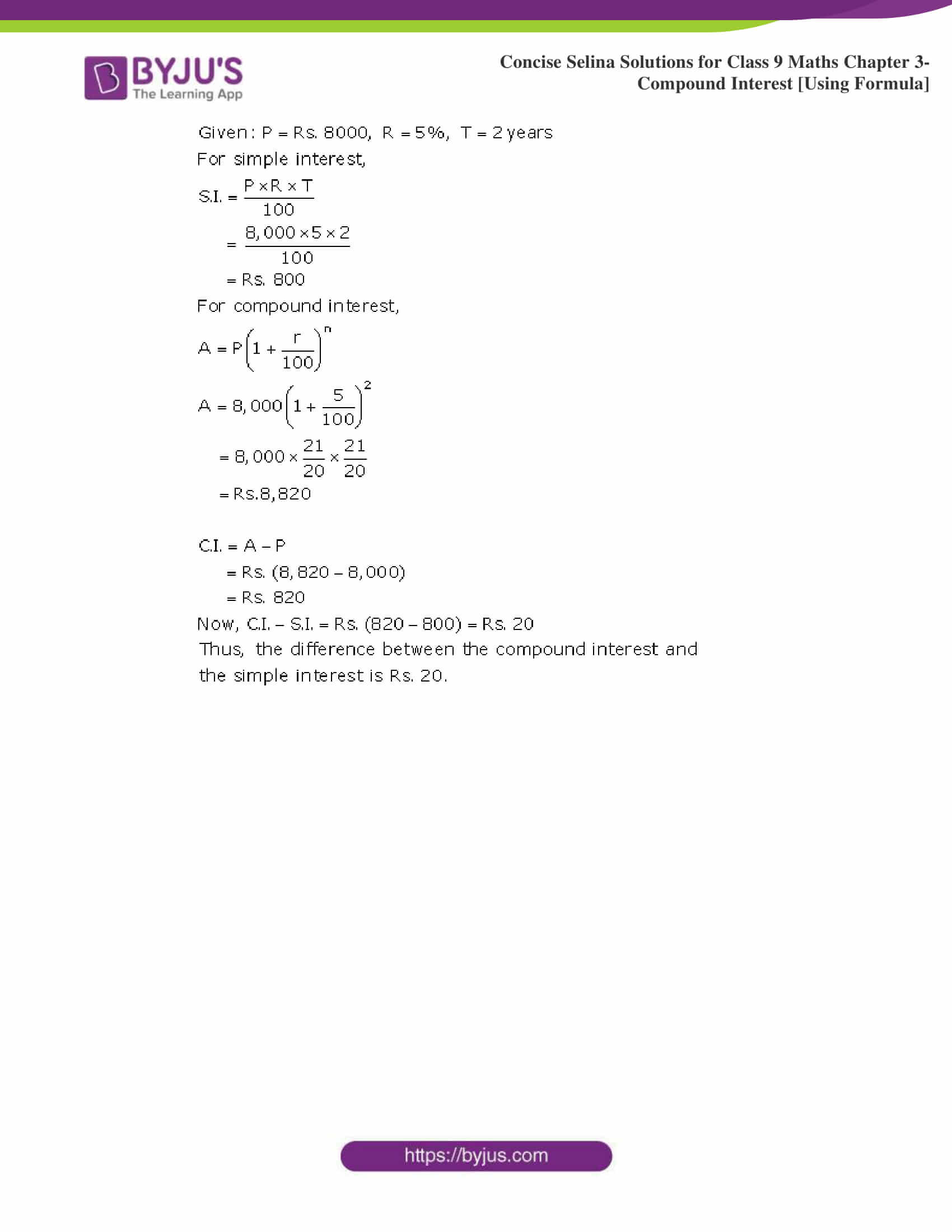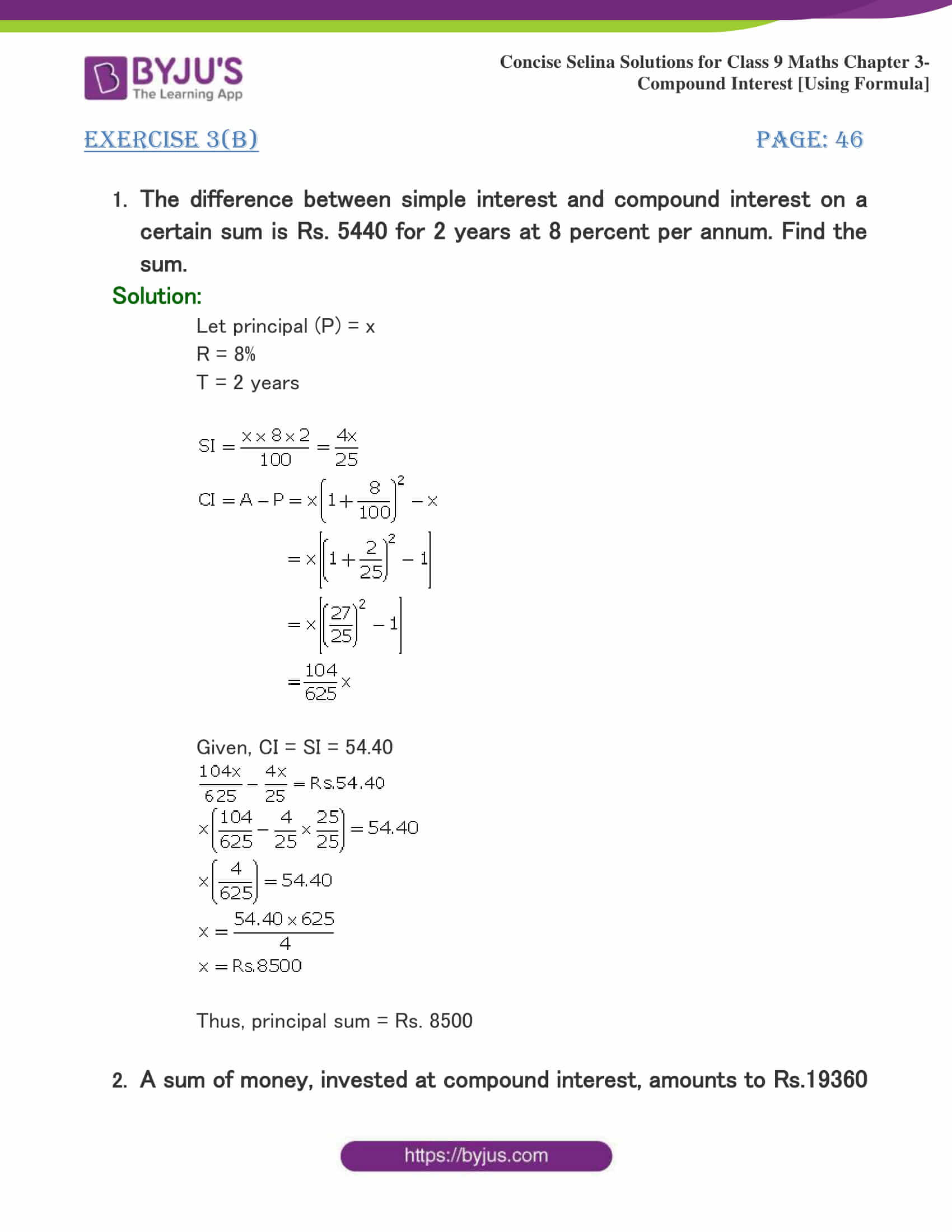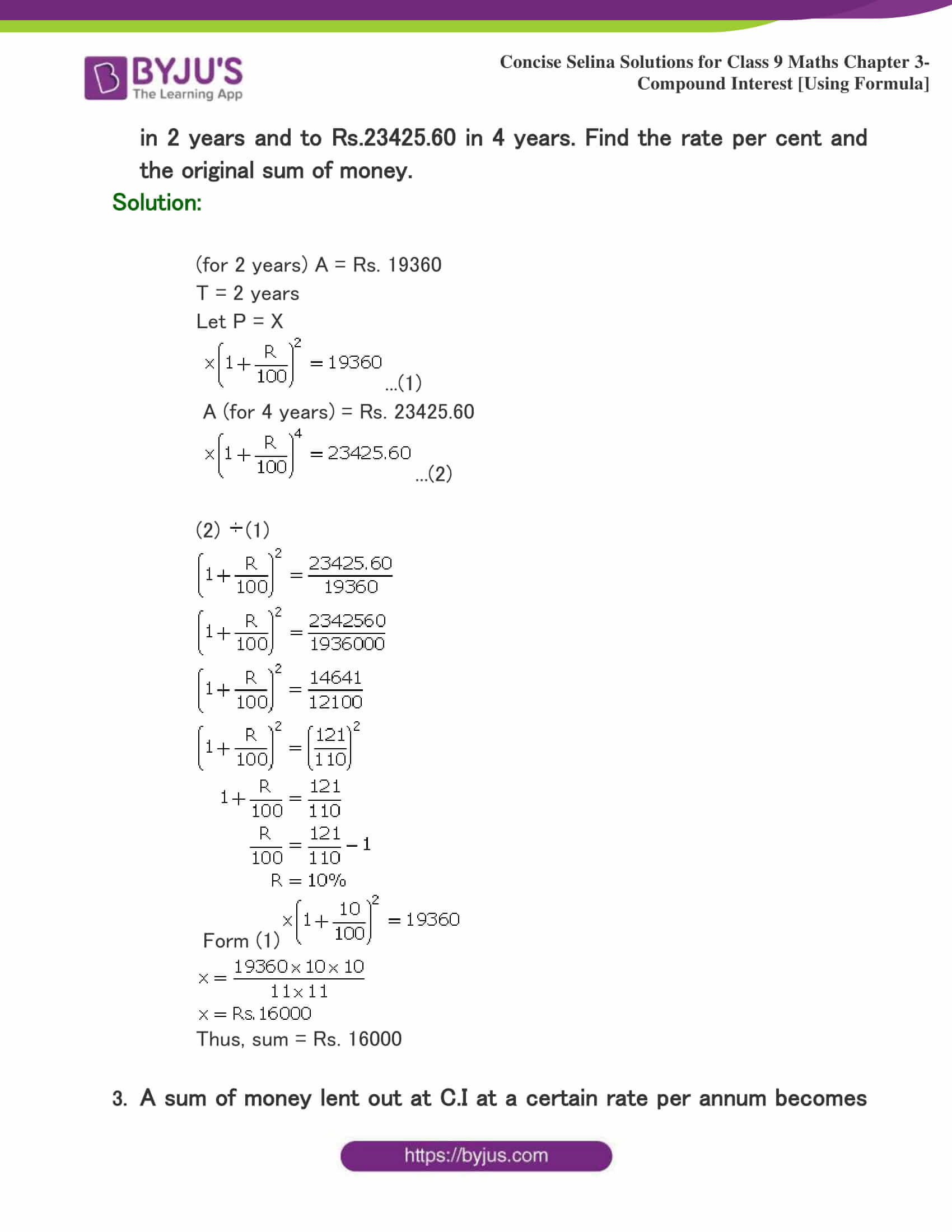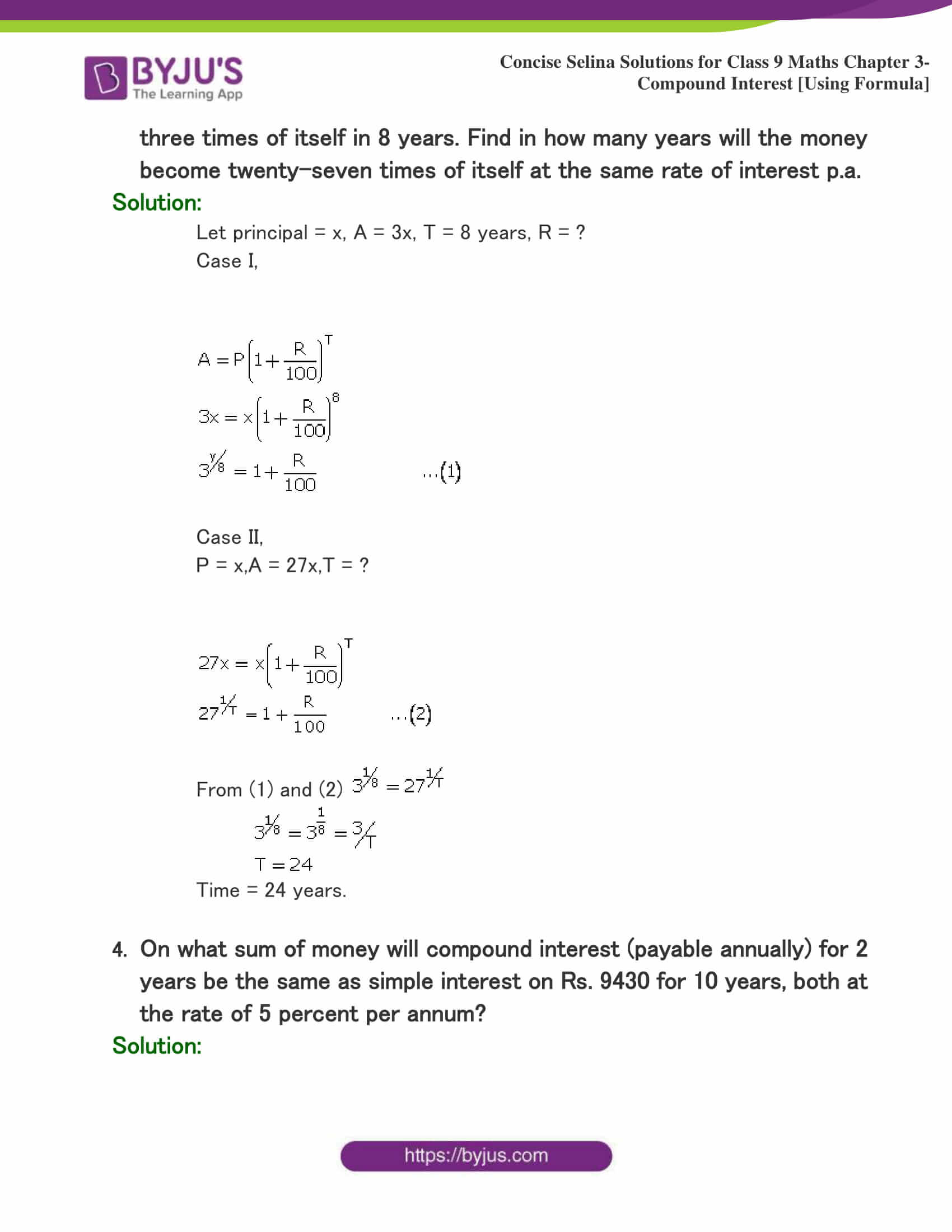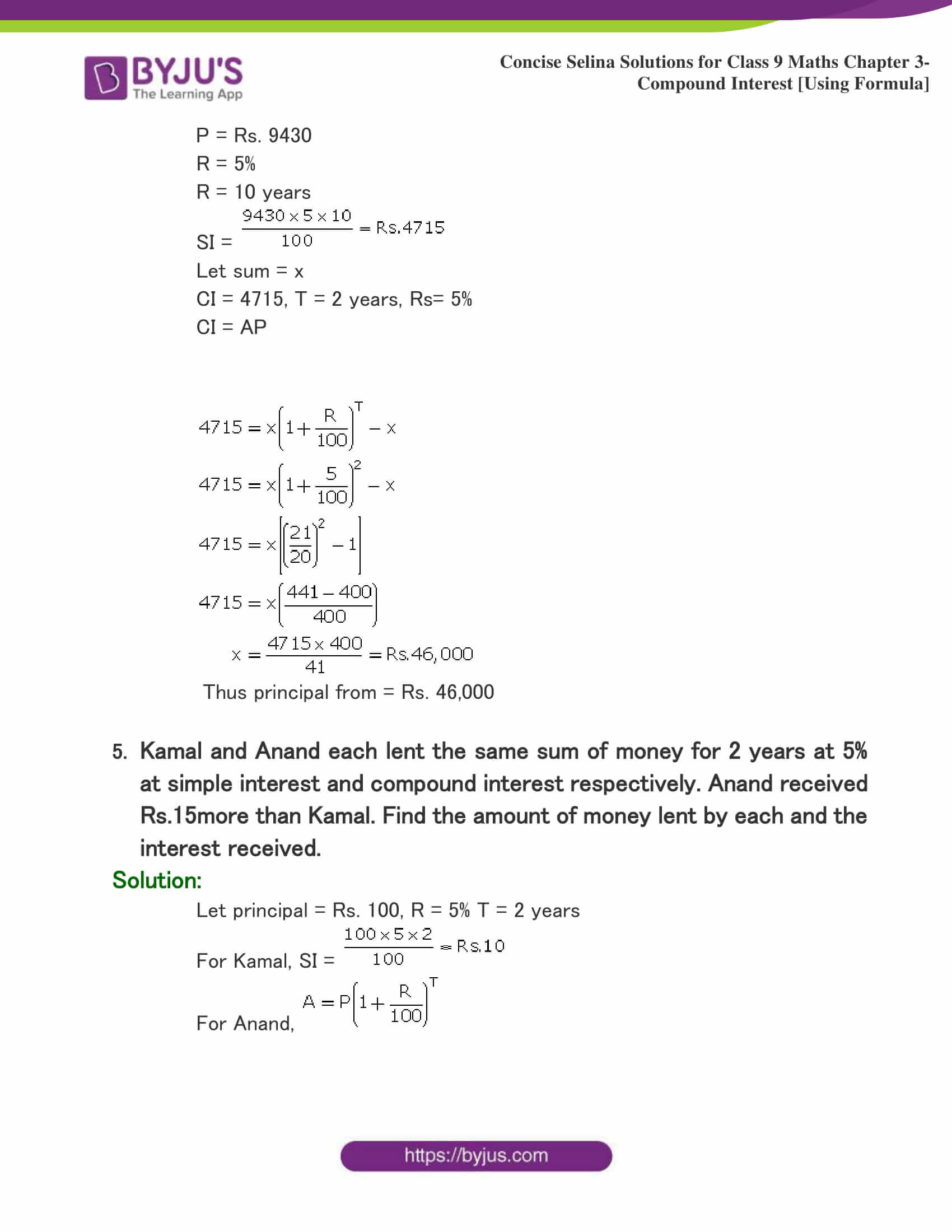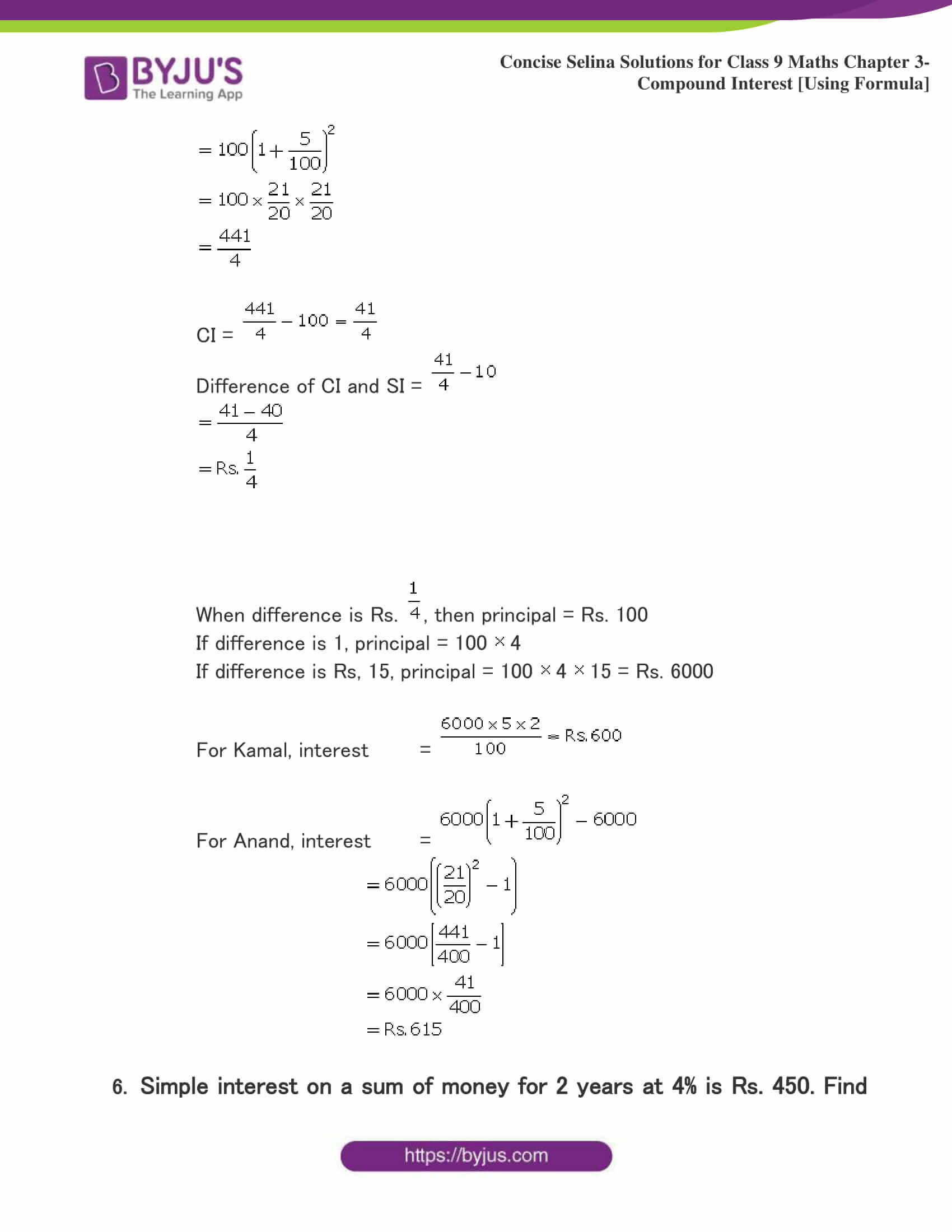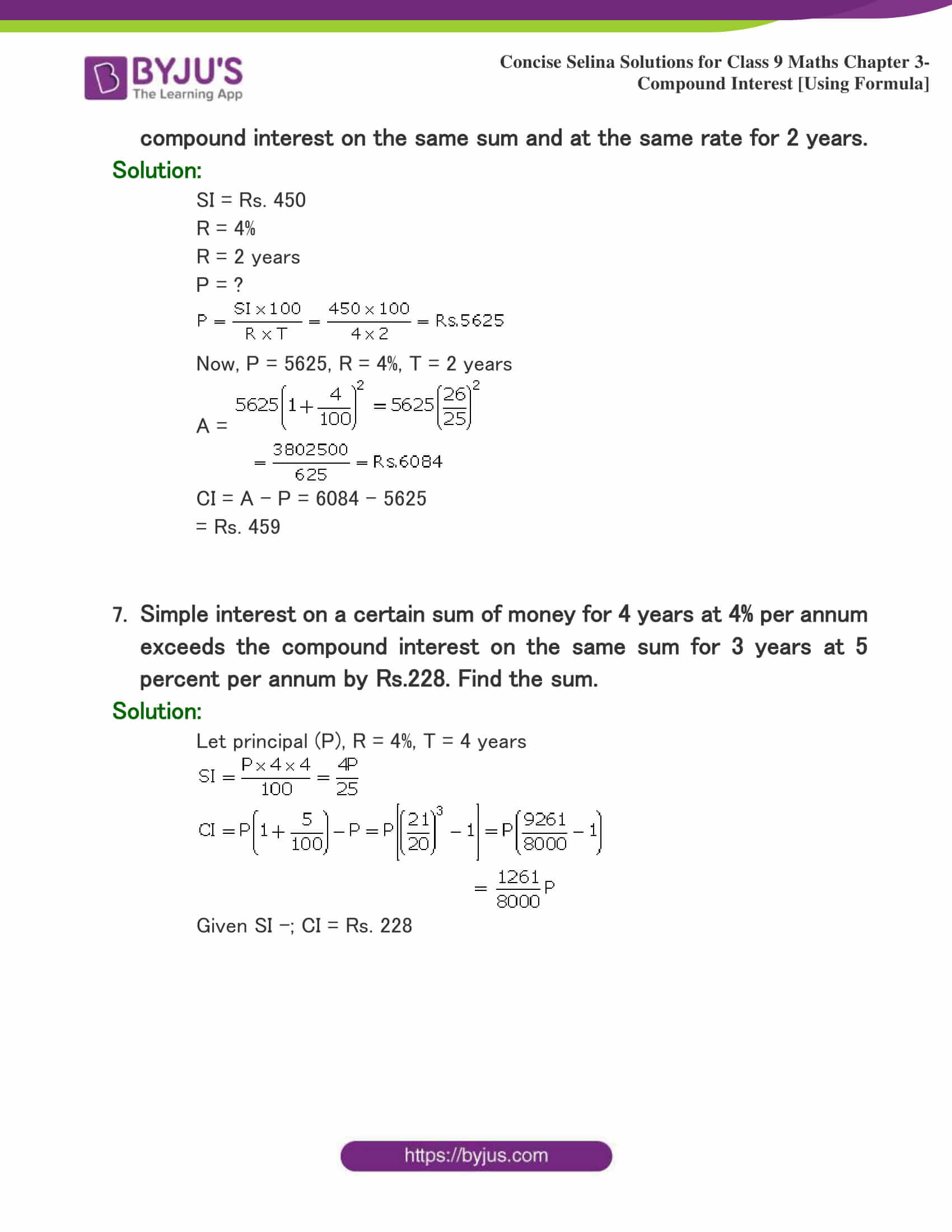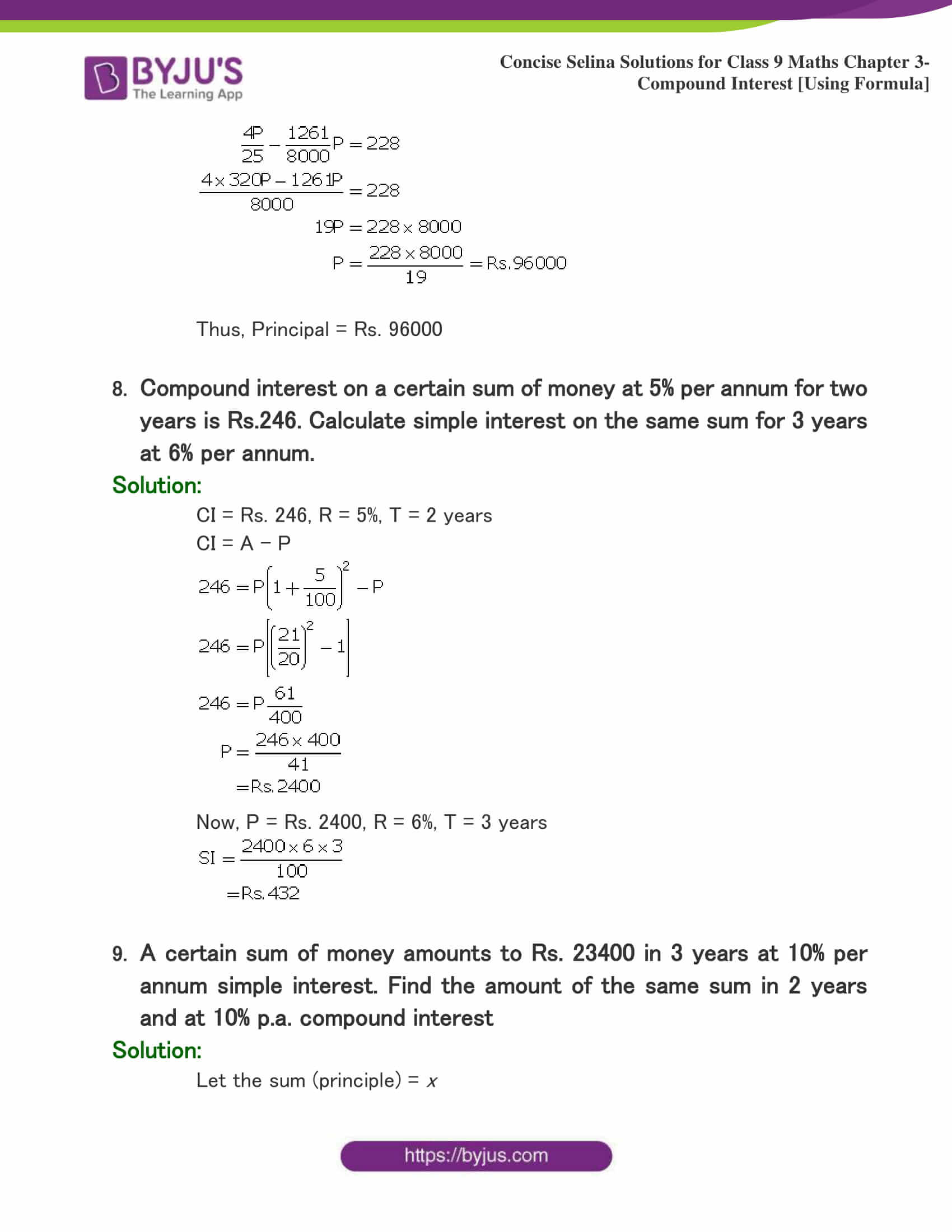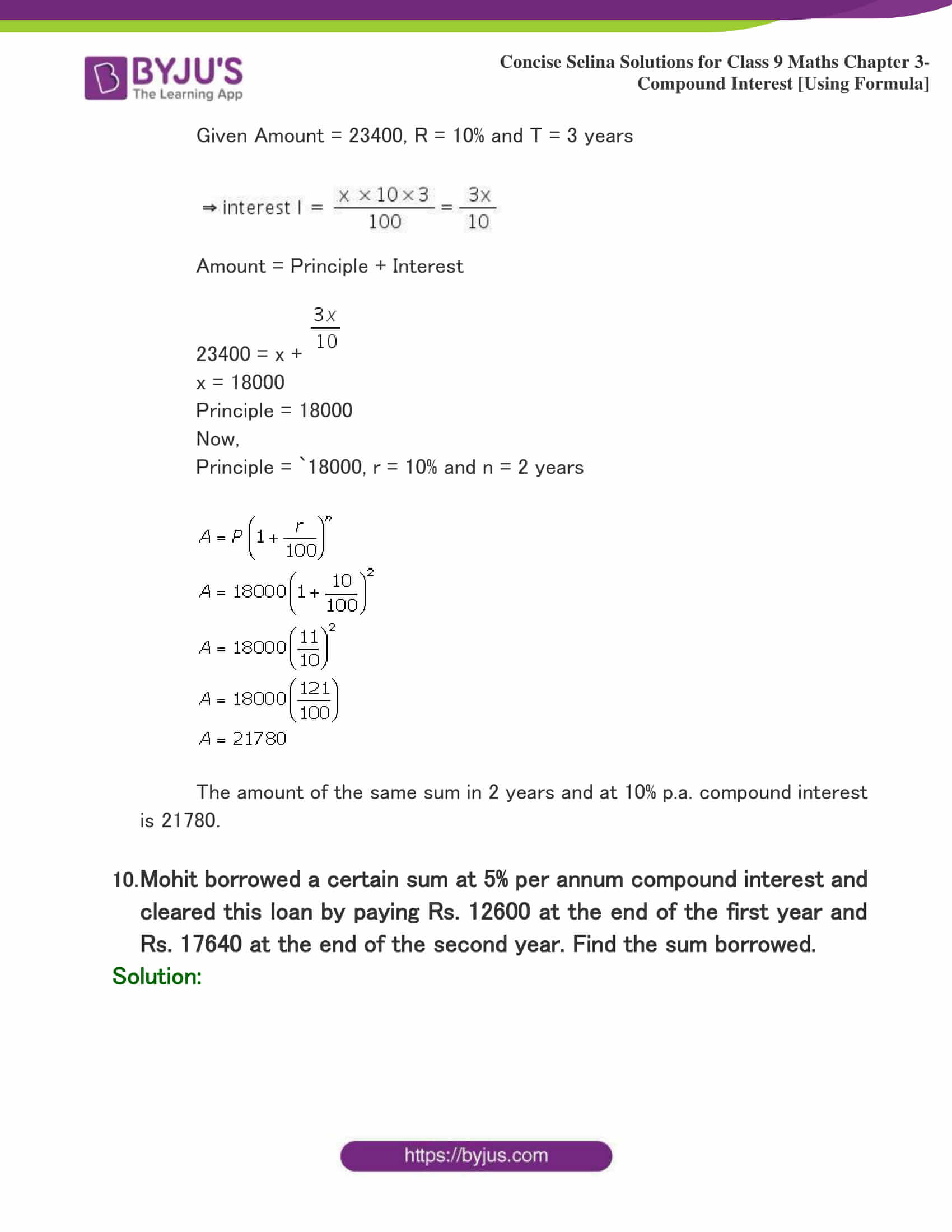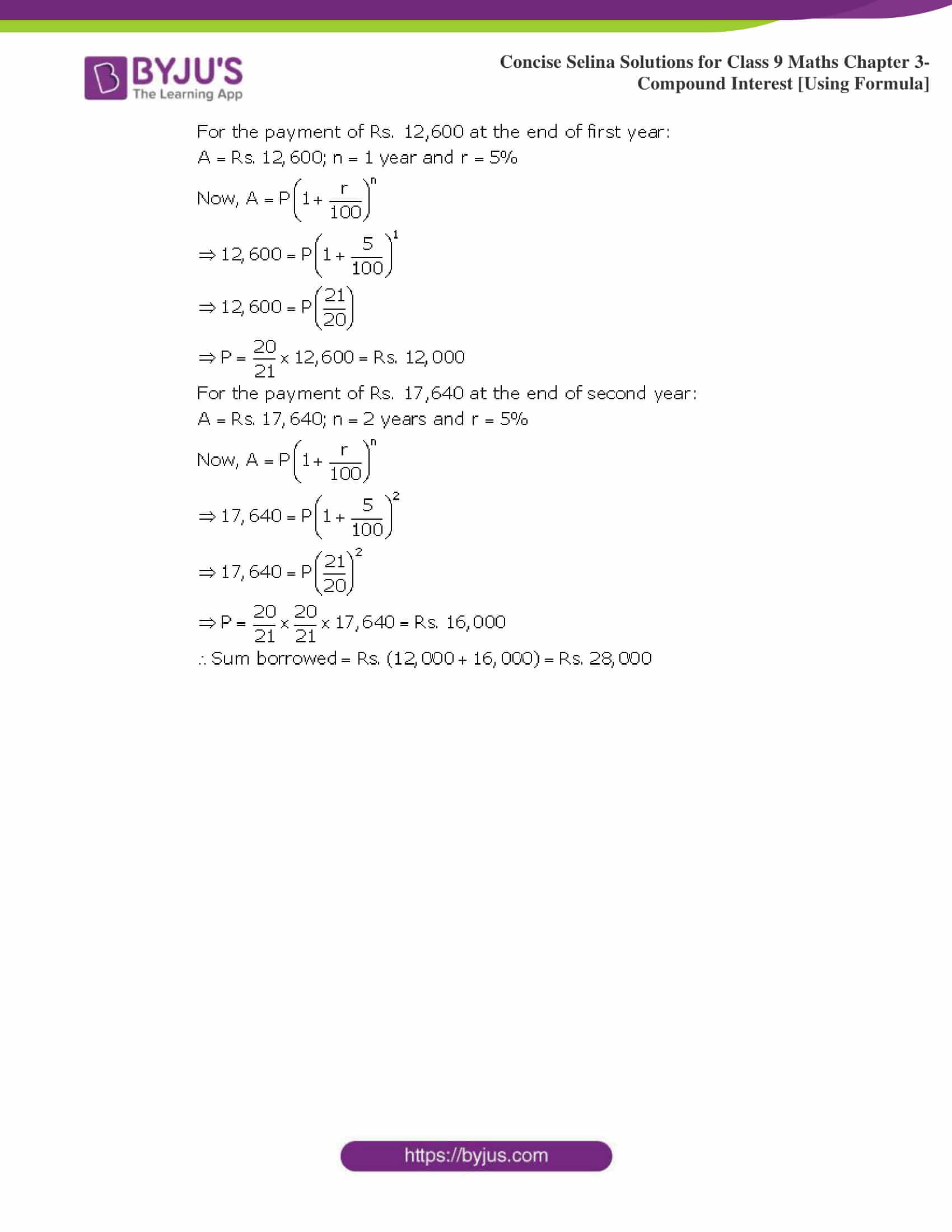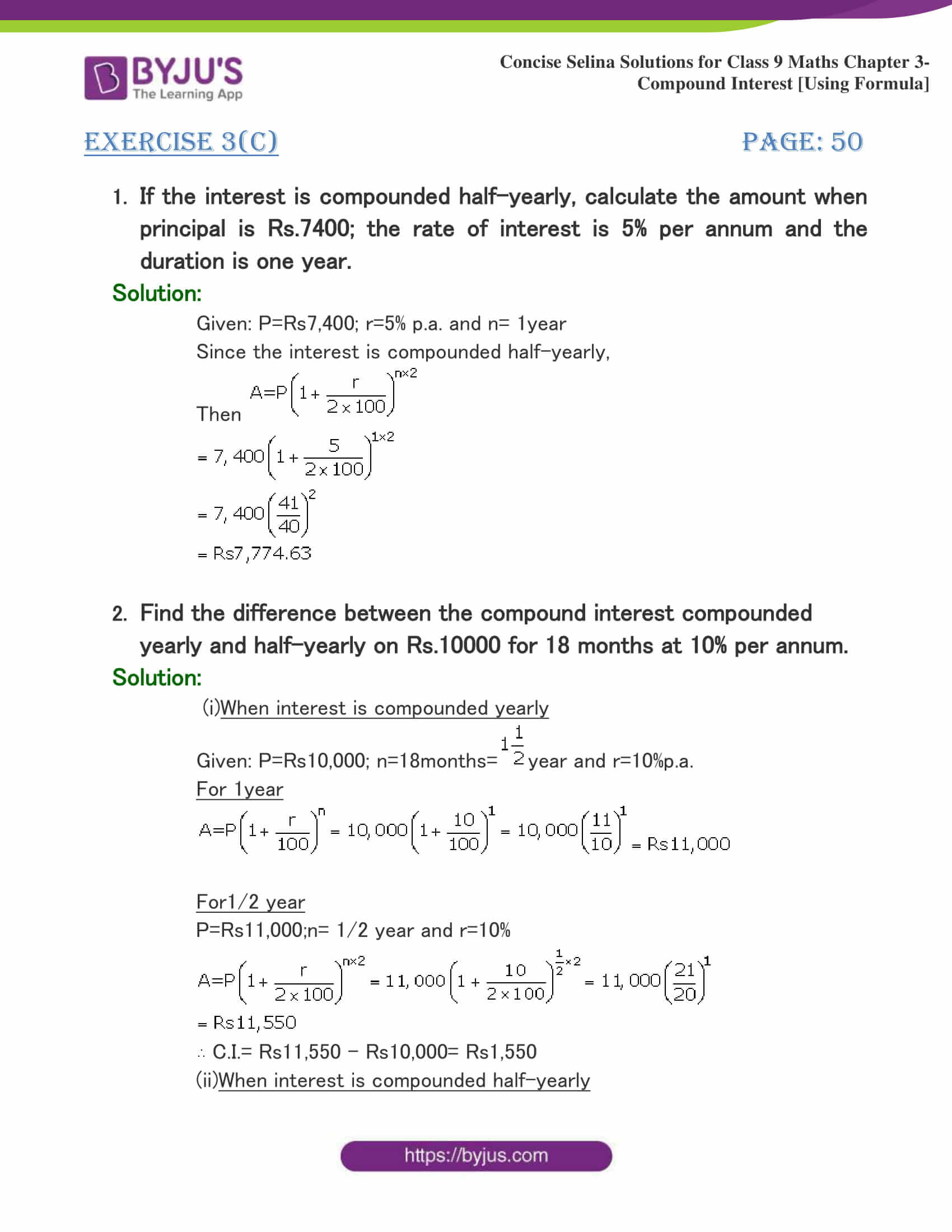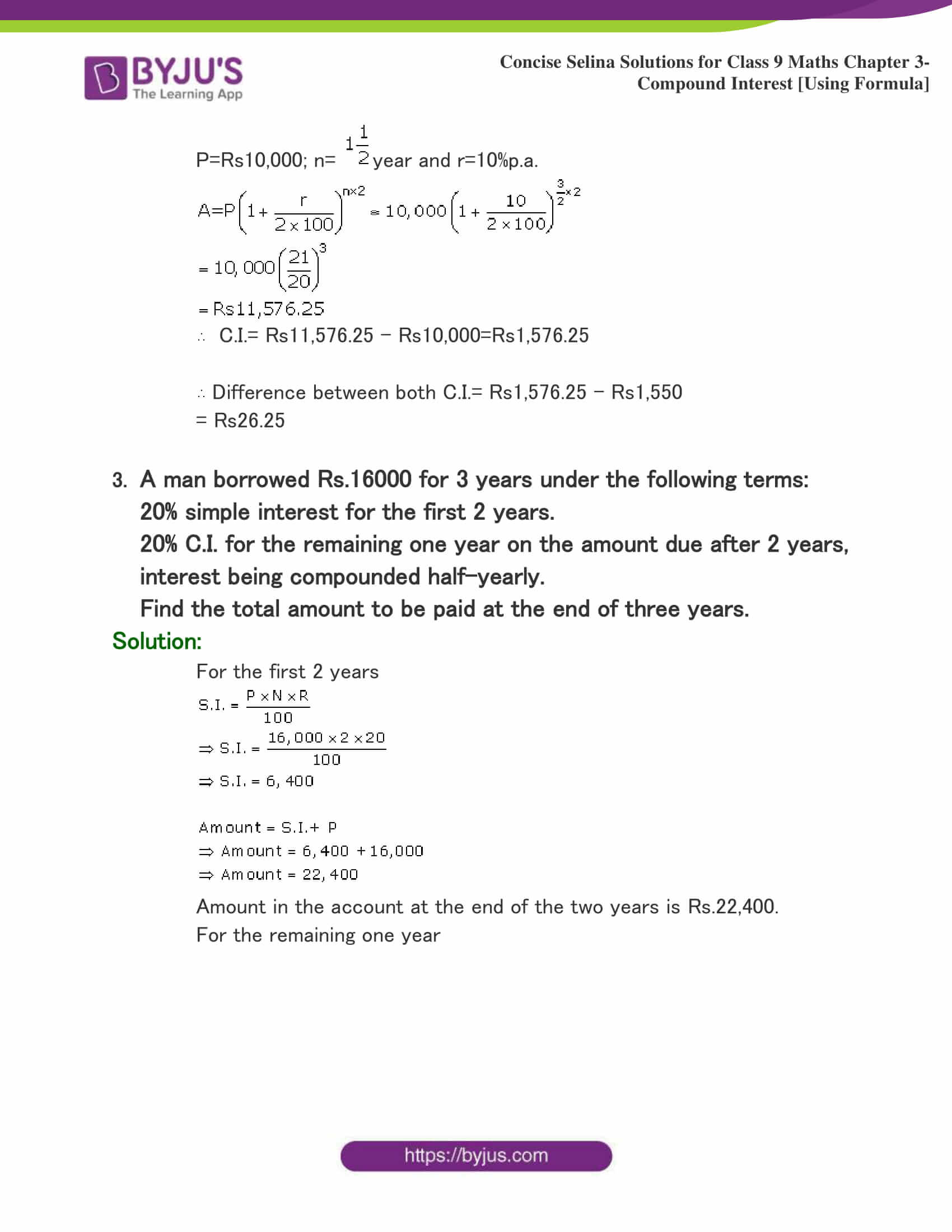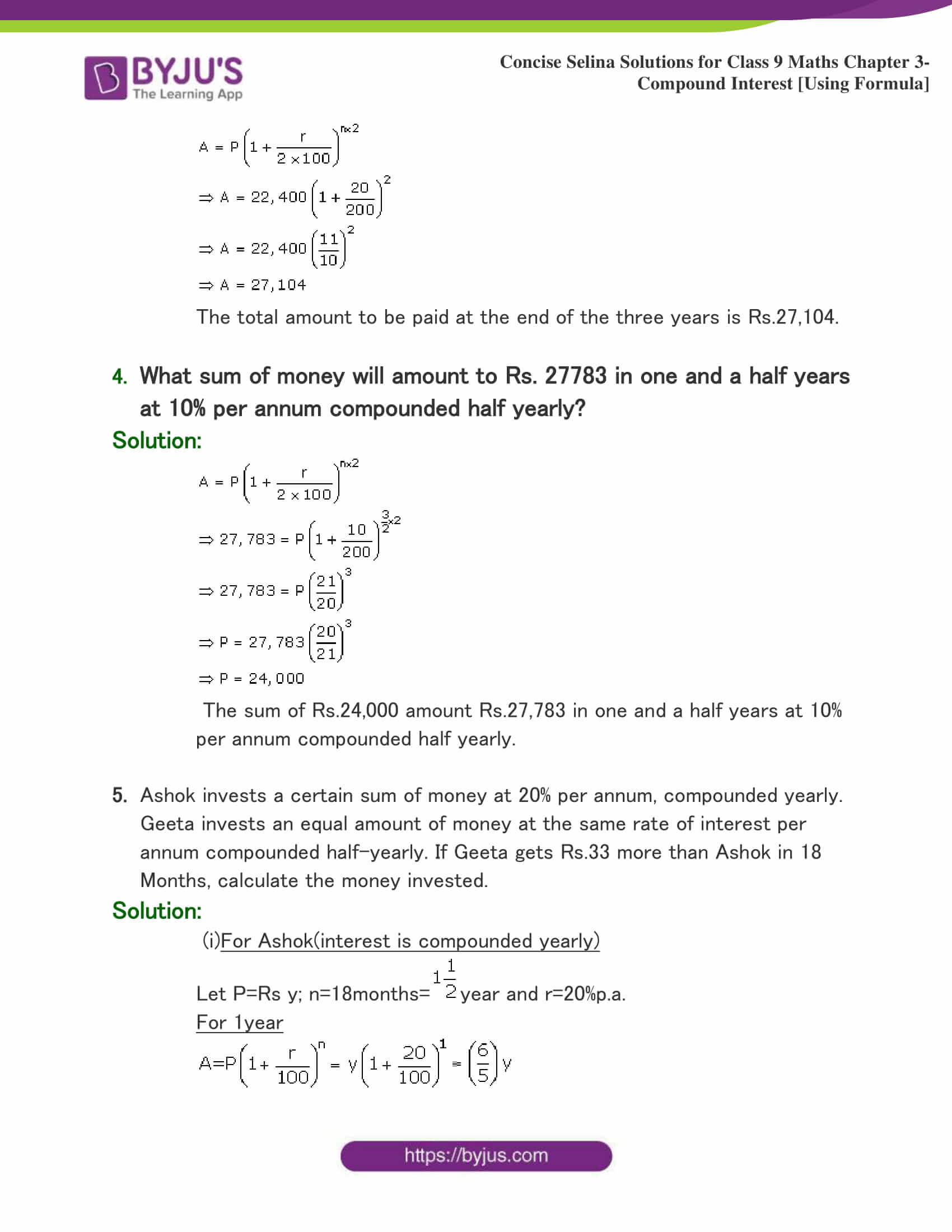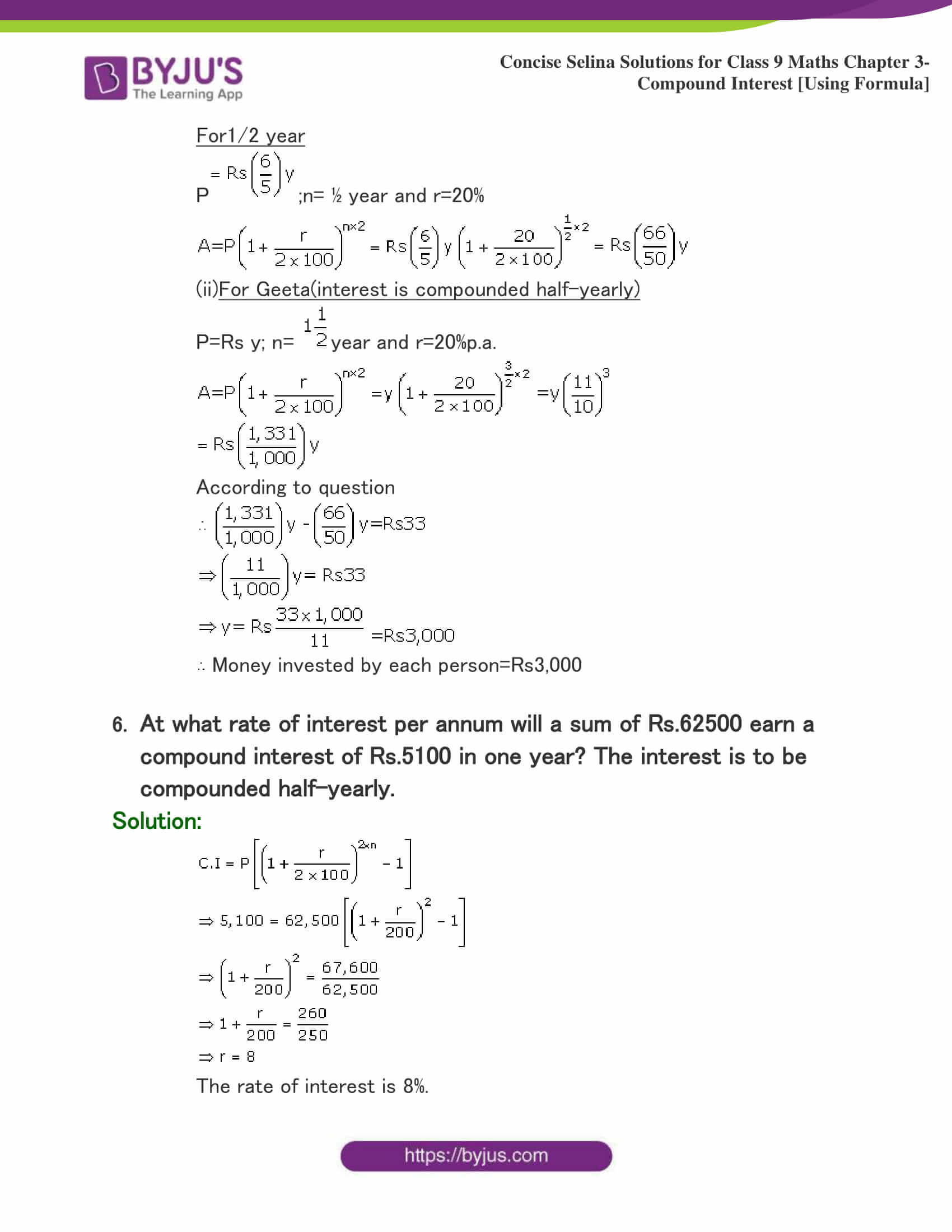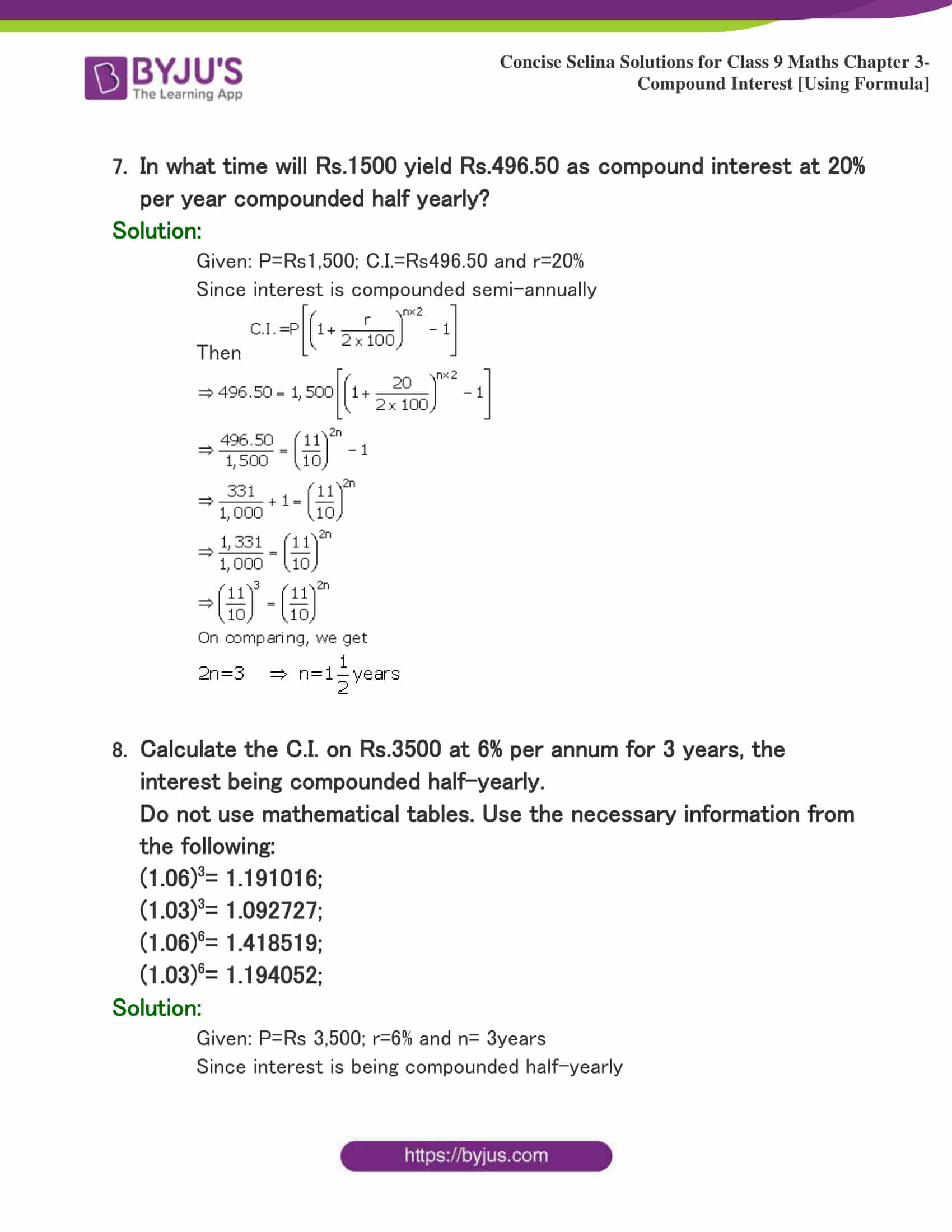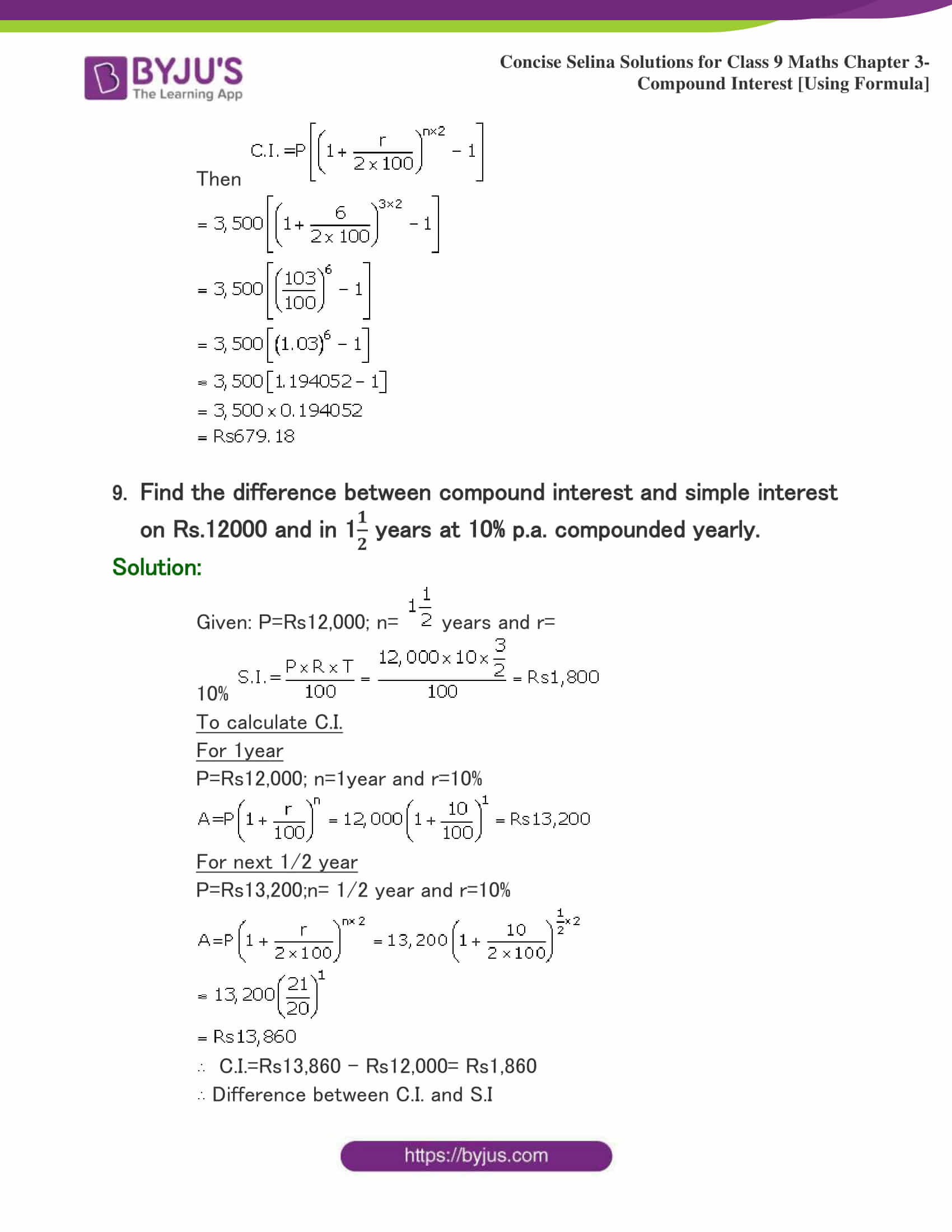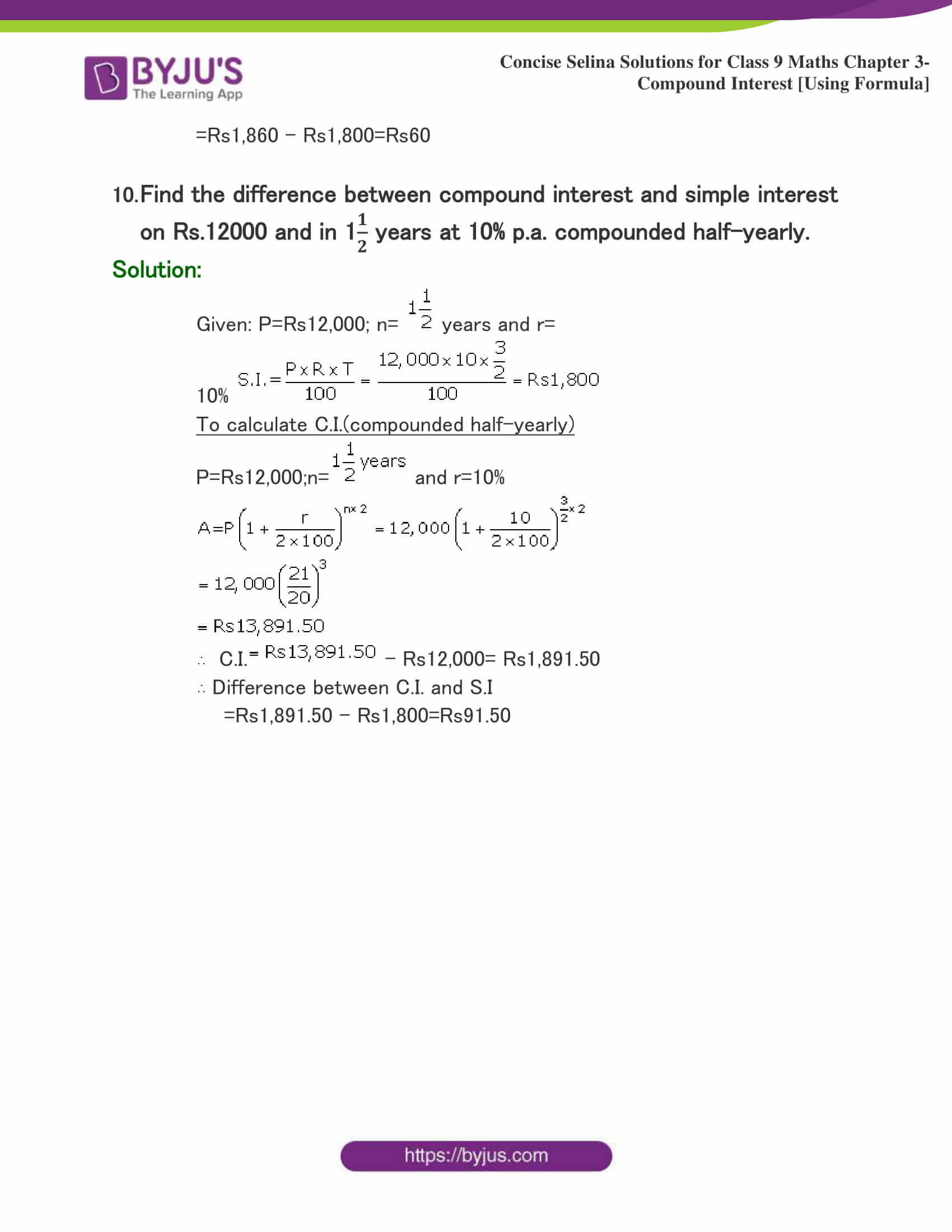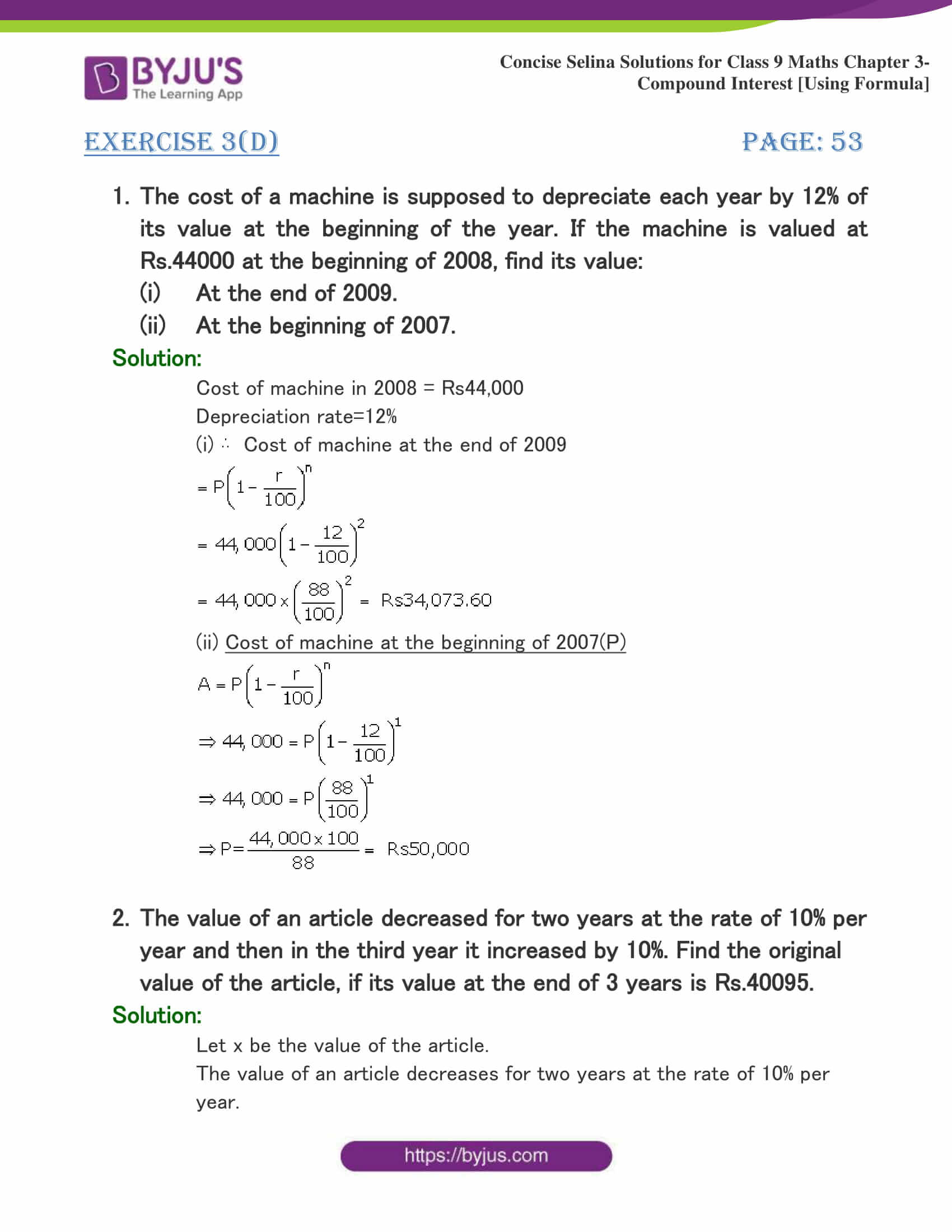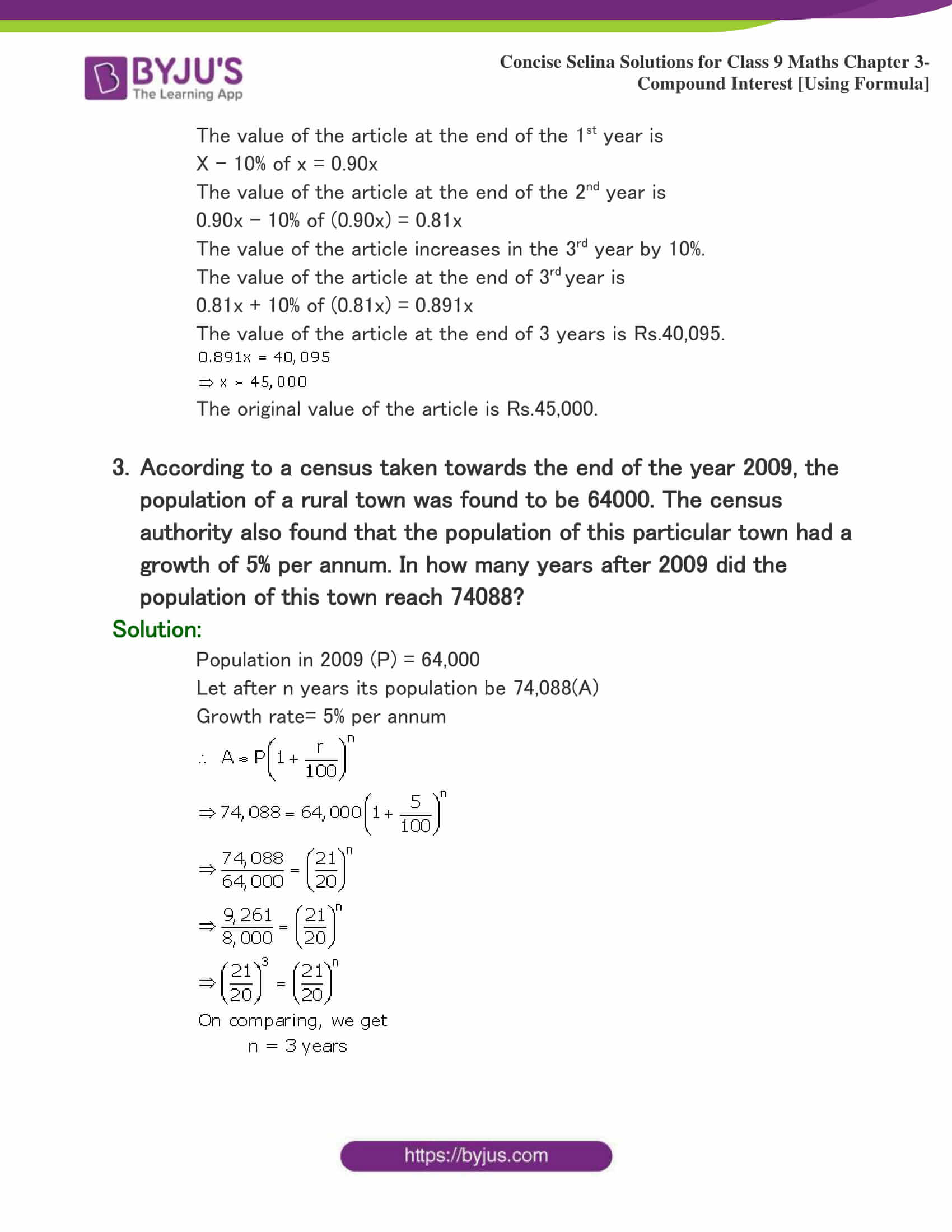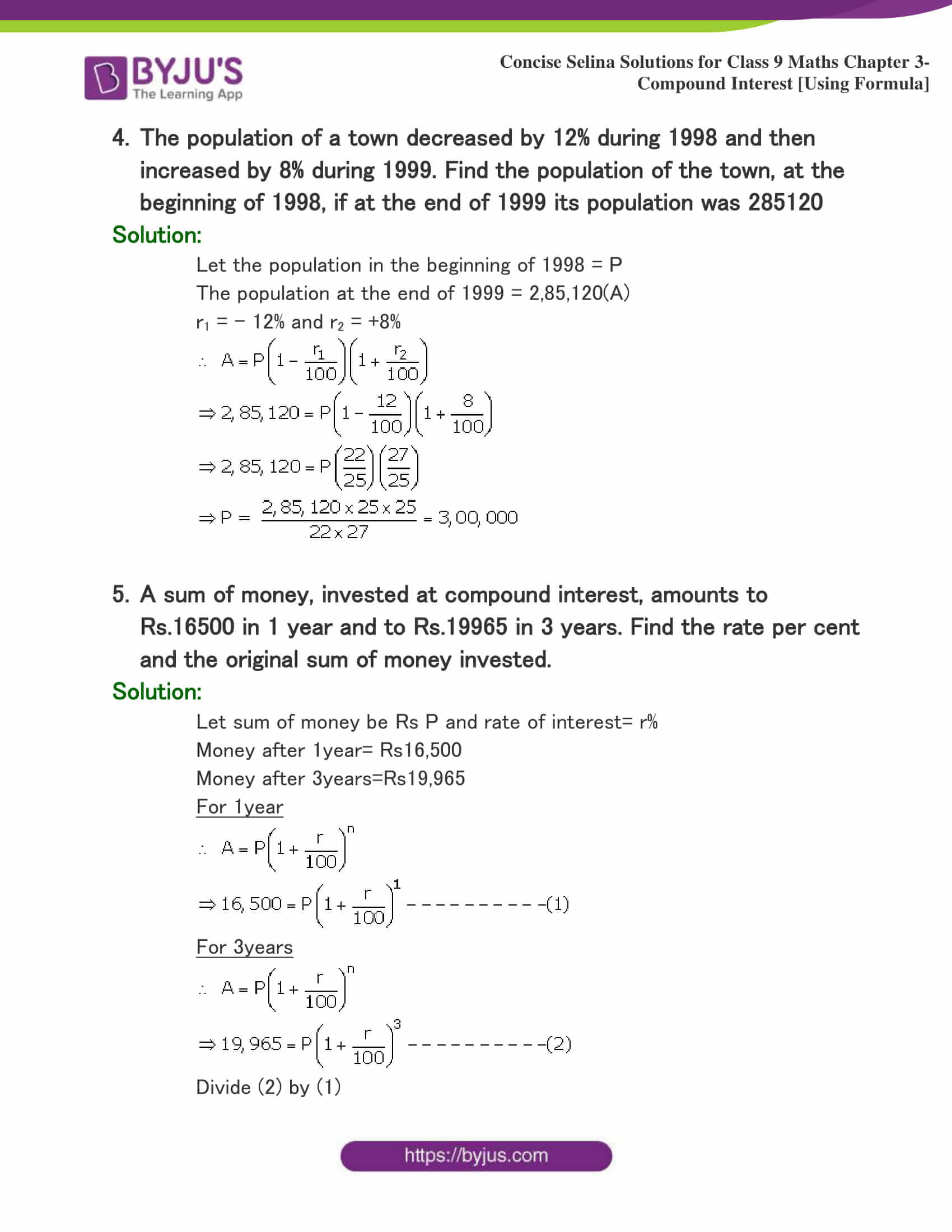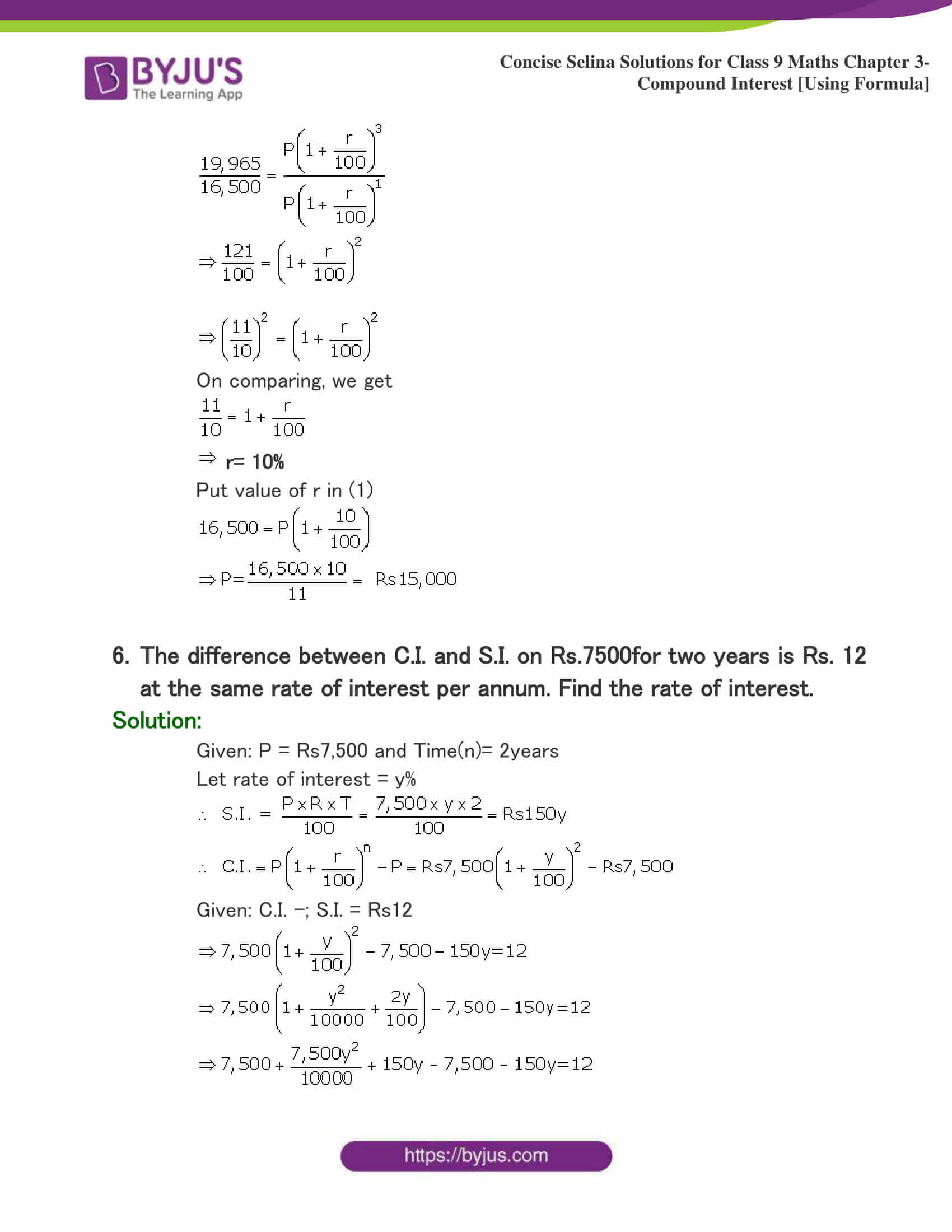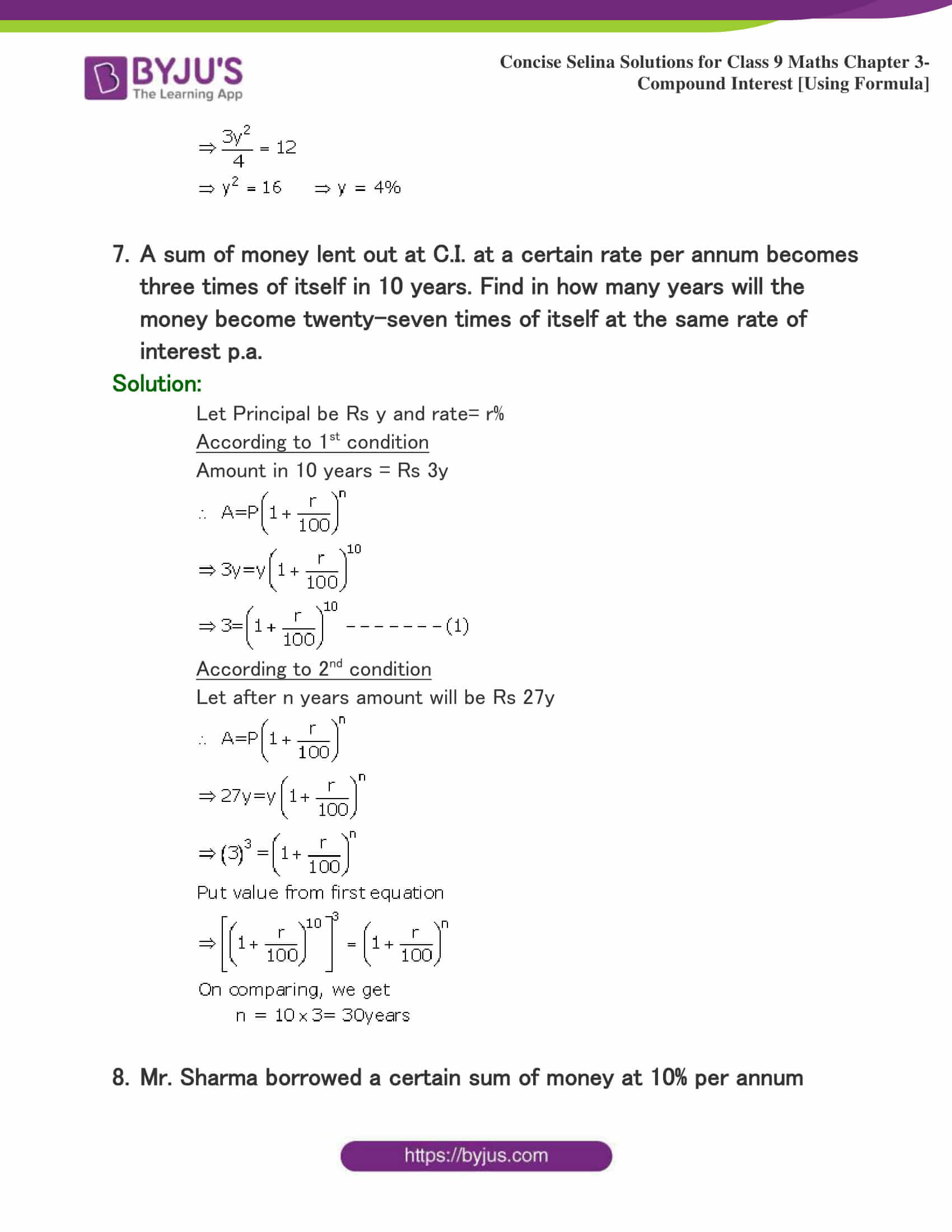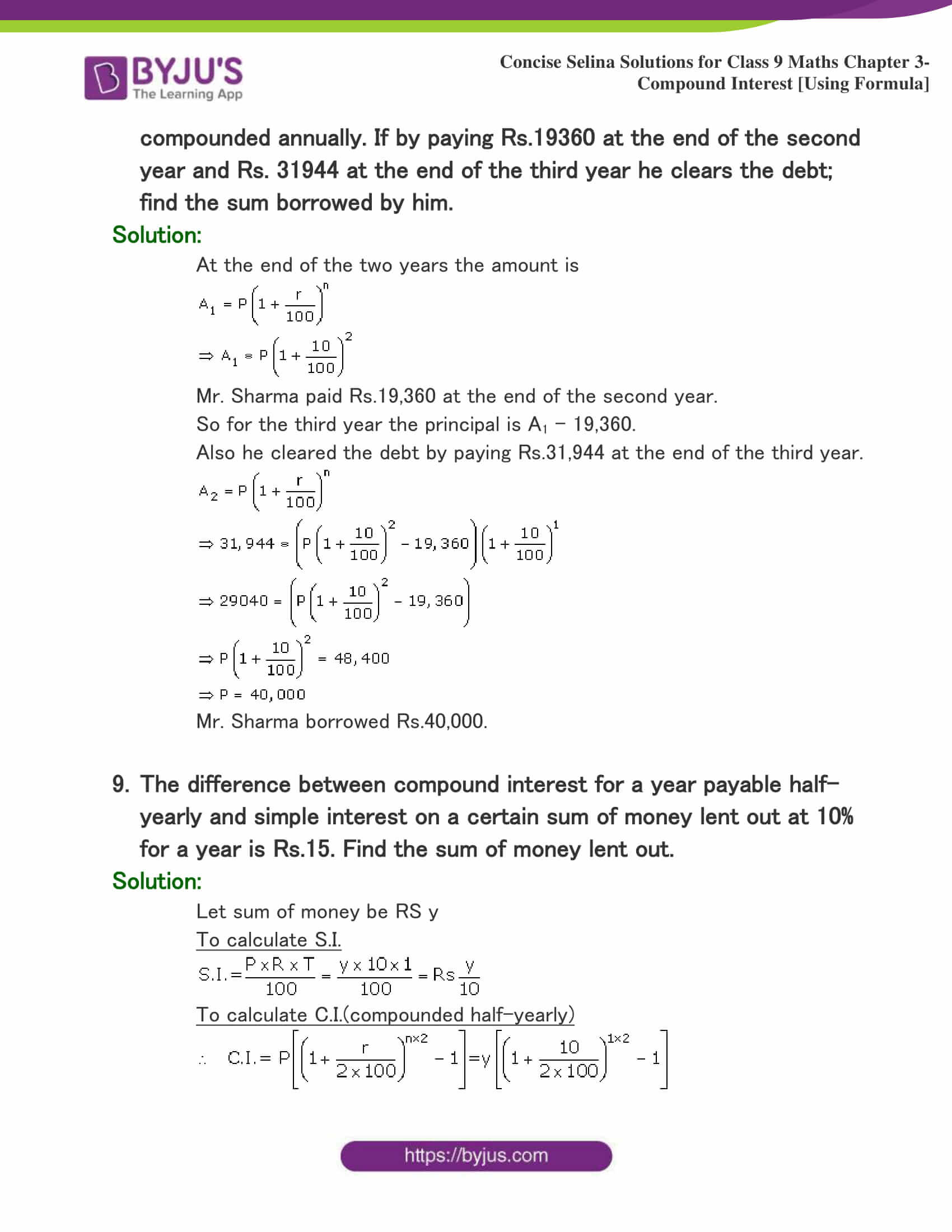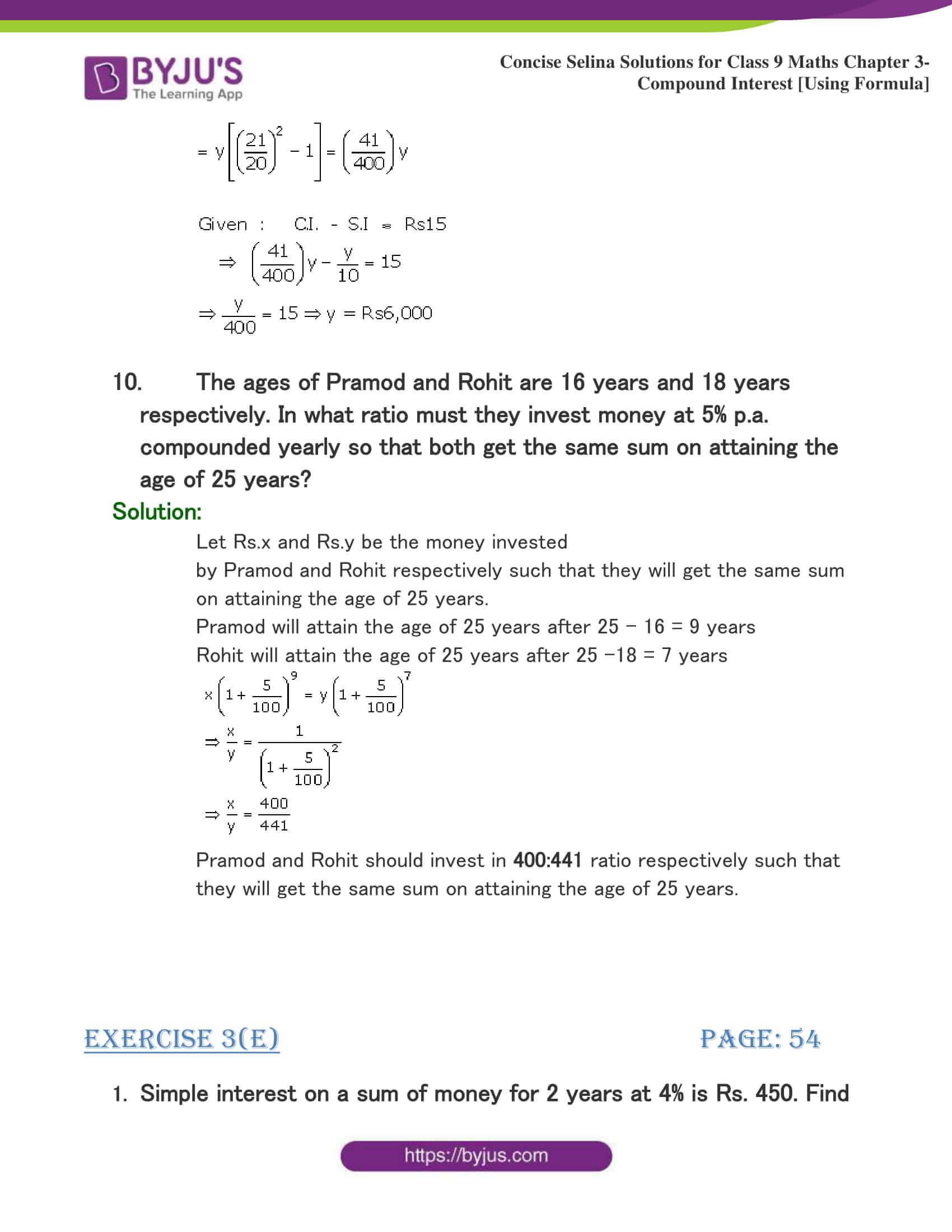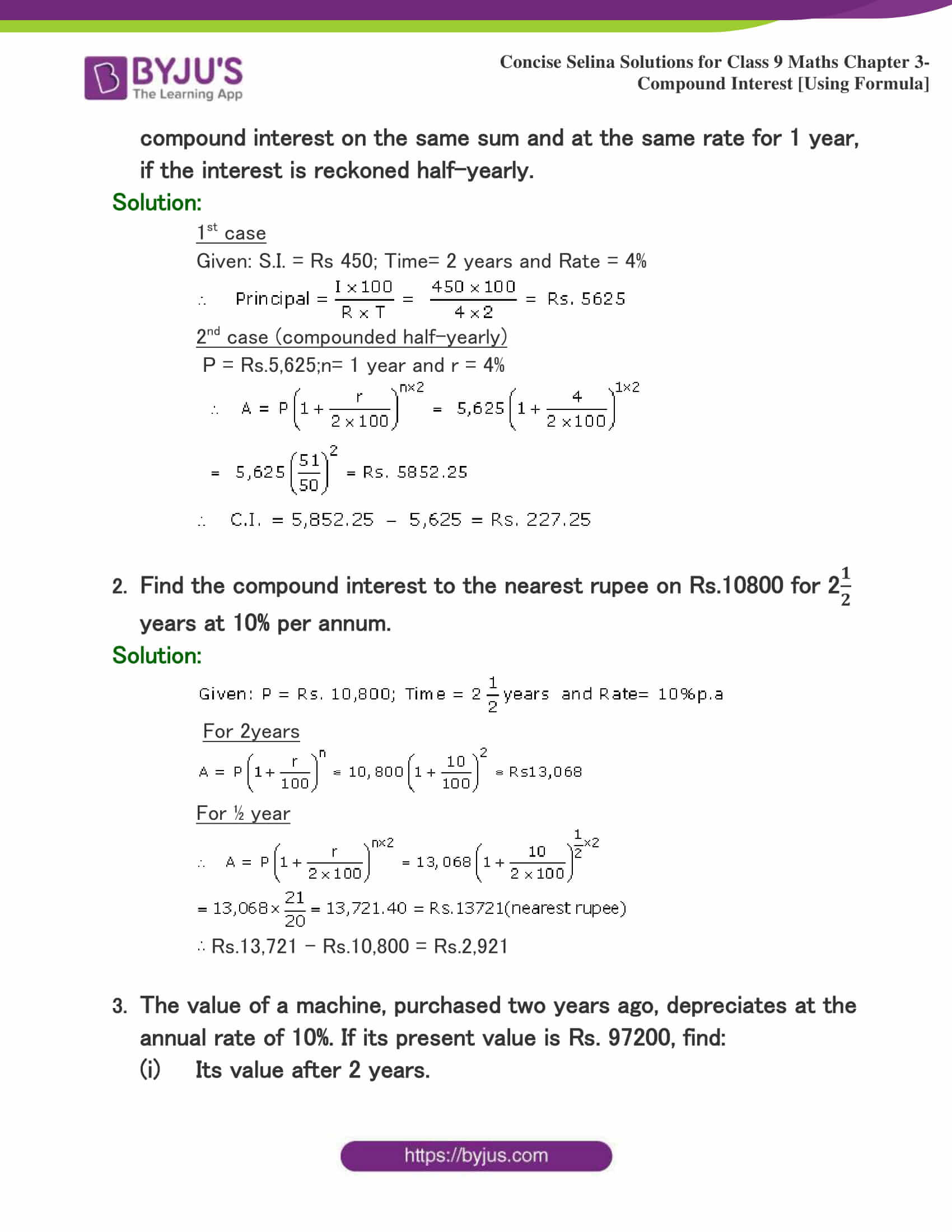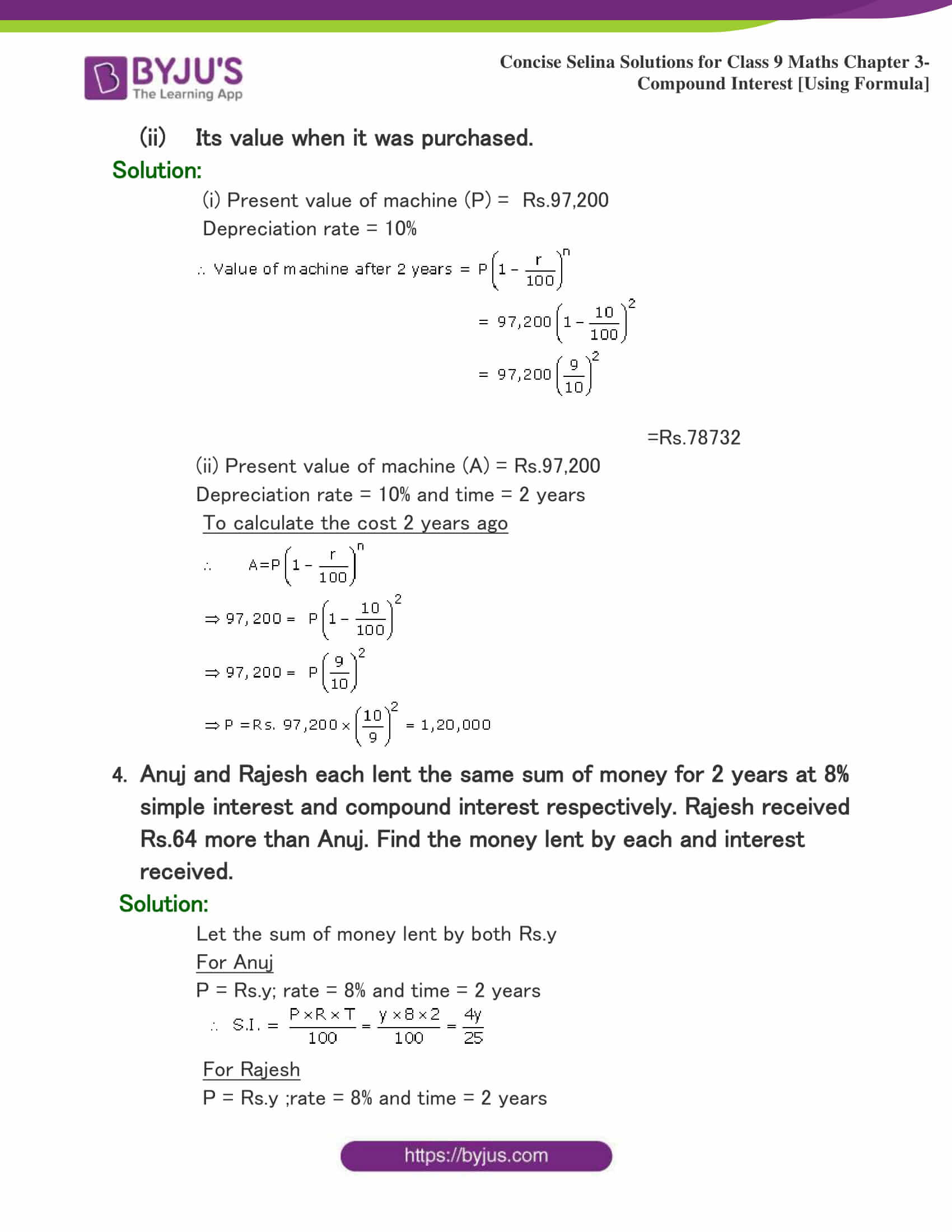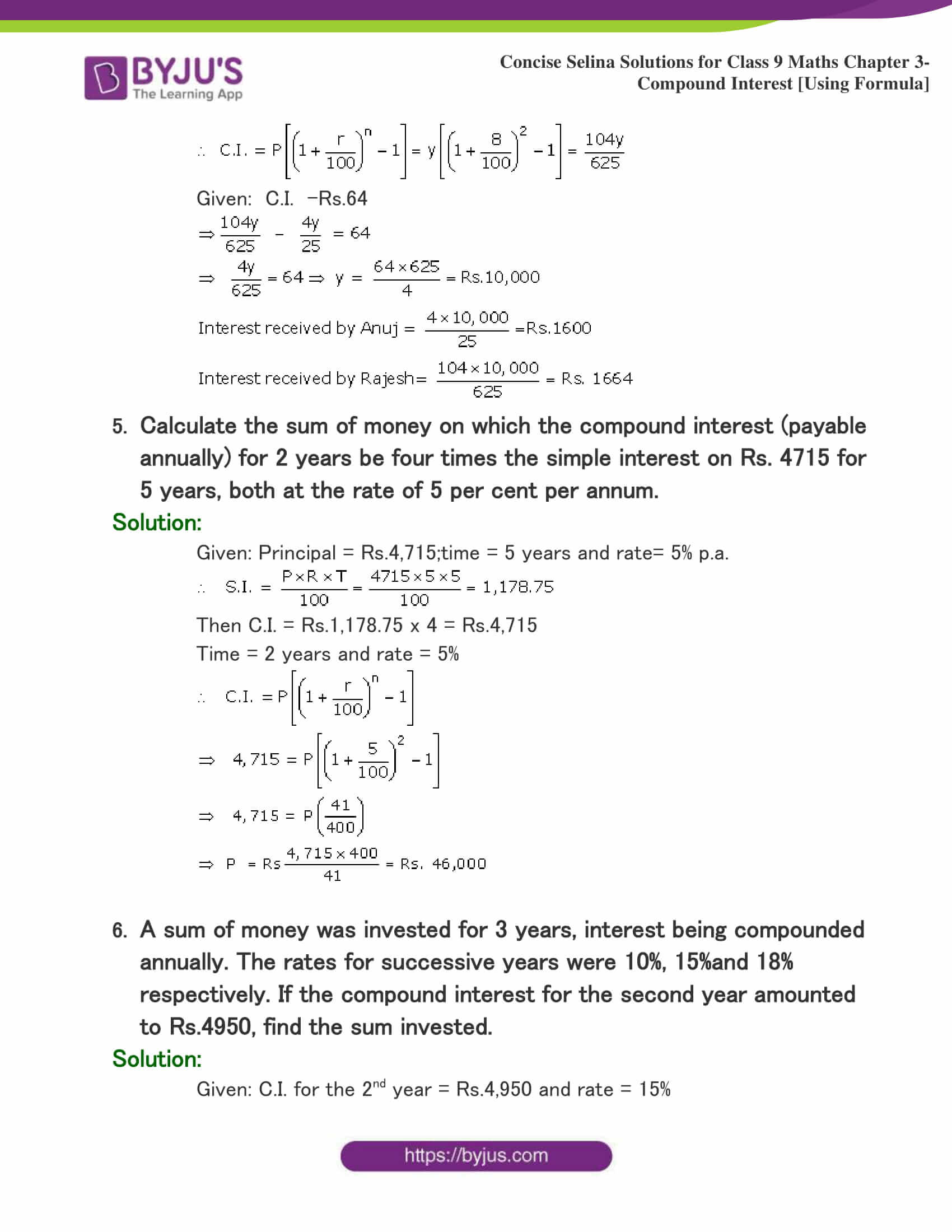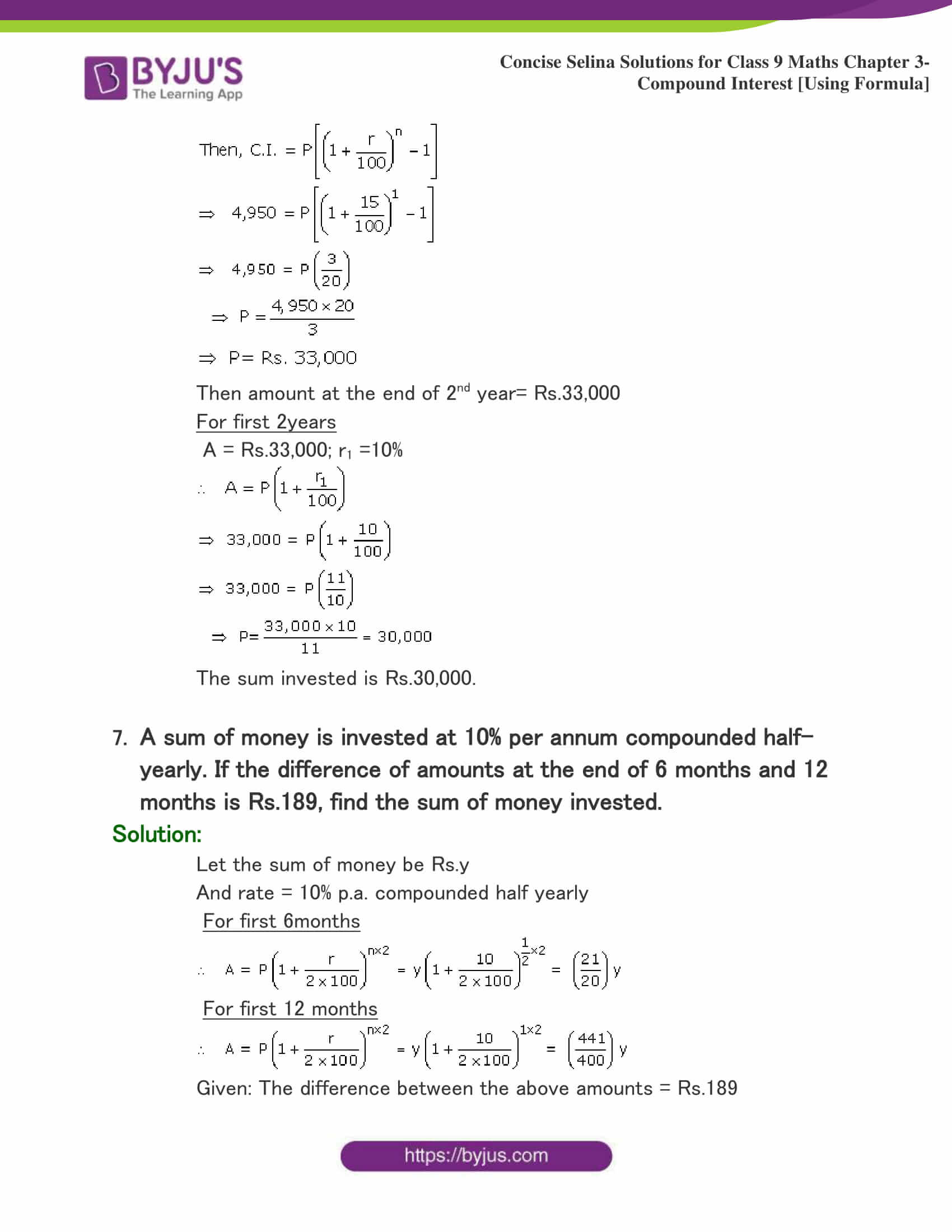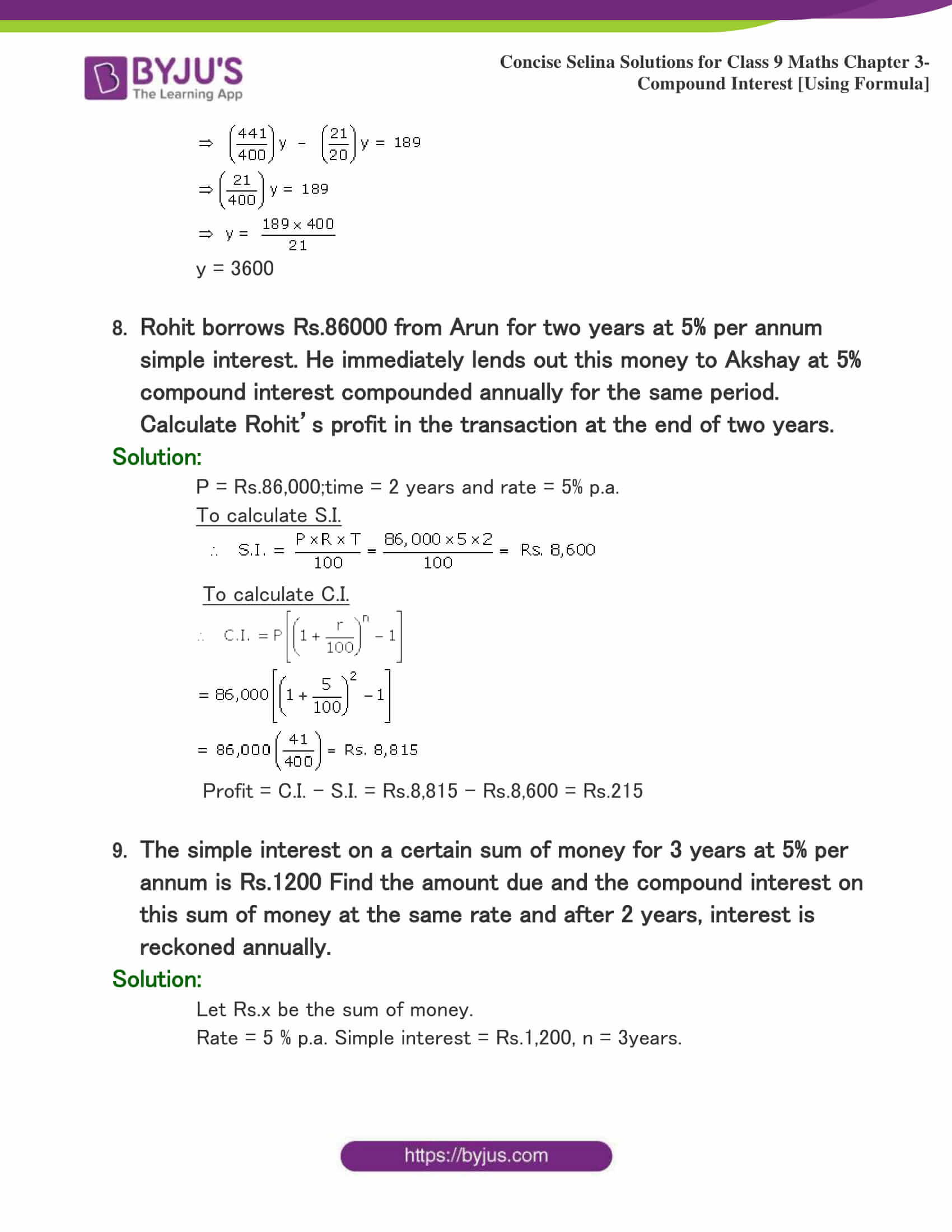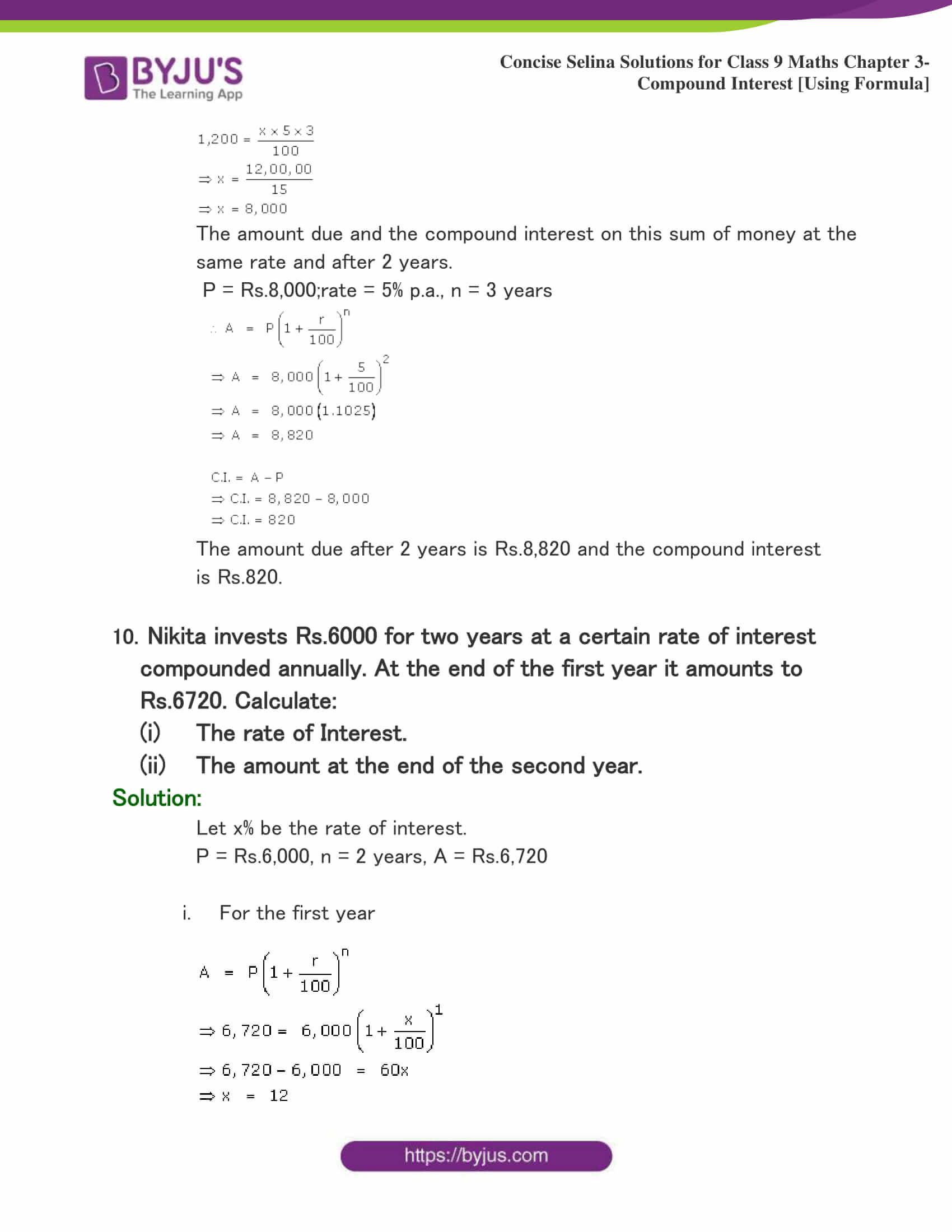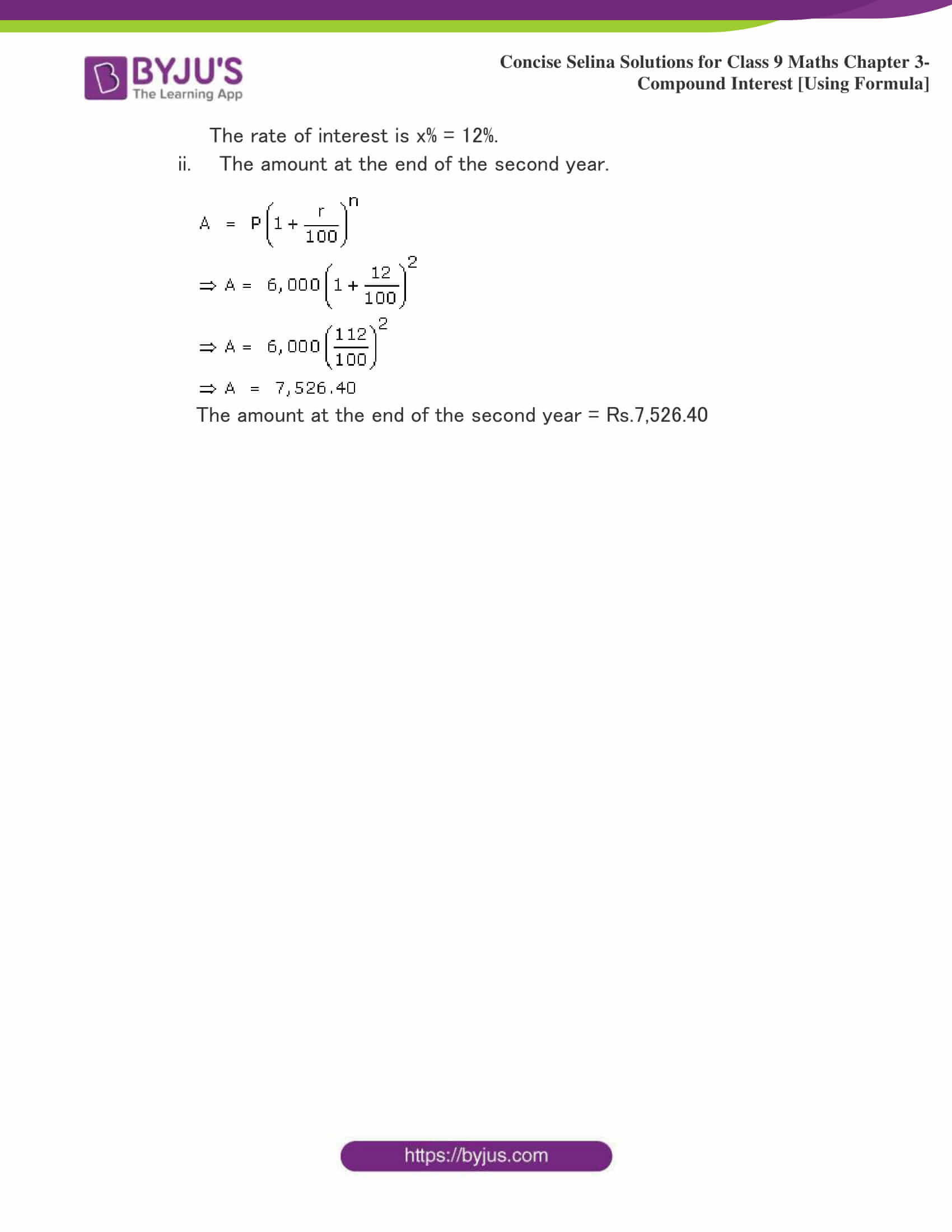## Selina Solutions for Class 9 Maths Chapter 3- Compound Interest [Using Formula]

The Chapter 3, Compound Interest [Using Formula], contains 5 exercises and the Solutions given here contains the answers for all the questions present in these exercises. Let us have a look at some of the topics that are being discussed in this chapter.

3.1 Introduction

3.2 Using Formula

3.3 Inverse Problems

3.4 Miscellaneous Problems

3.5 When the Interest Is Compounded Half-Yearly (Two Times in a Year)

3.6 When the Time is Not an Exact Number of Years And the Interest is Compounded Yearly

3.7 Other Applications of the Formula

## Selina Solutions for Class 9 Maths Chapter 3- Compound Interest [Using Formula]

The Chapter 3 of class 9 is a continuation of chapter 2. In chapter 2 the students were taught to calculate the compound interest as a repeated simple interest computation with a growing principal. This calculation becomes tedious as the number of conversion period increases. To make the calculation of compound interest easier, certain formulae are used. Read and learn the Chapter 3 of Selina textbook to get to know more about Compound Interest [Using Formula]. Learn the Selina Solutions for Class 9 effectively to attain excellent result in the examination.